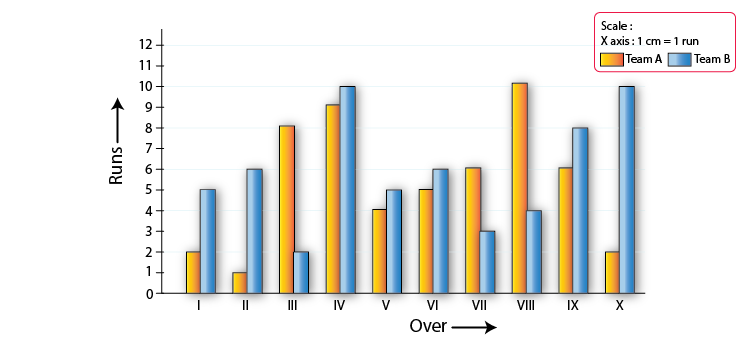# RD Sharma Solutions for Class 8 Maths Chapter 27 Introduction to Graphs

In our day-to-day lives, we come across many situations like the temperature of a patient taken at different times of a day, sales of a shopkeeper on various days of the week, etc. In this chapter, we shall discuss the construction and reading of graphs. In order to draw graphs, we first plot various points and then they are joined by a freehand curve. Students who wish to have strong command over the subject can refer to RD Sharma Class 8. This resource can be used by the students to prepare for their exams as well. From the exam point of view, the solutions are designed by our experts in simple language which helps in better understanding of concepts. The solutions of RD Sharma Class 8 are available in the pdf format, which can be downloaded from the links given below.

Chapter 27 Introduction to Graphs, contains two exercises and the RD Sharma Class 8 Solutions present in this page provide solutions to the questions present in each exercise. Now, let us have a look at the concepts discussed in this chapter.

• Cartesian plane.
• Plotting of points.
• Construction of graphs.

## Download the Pdf of RD Sharma Solutions for Class 8 Maths Chapter 27 Introduction to Graphs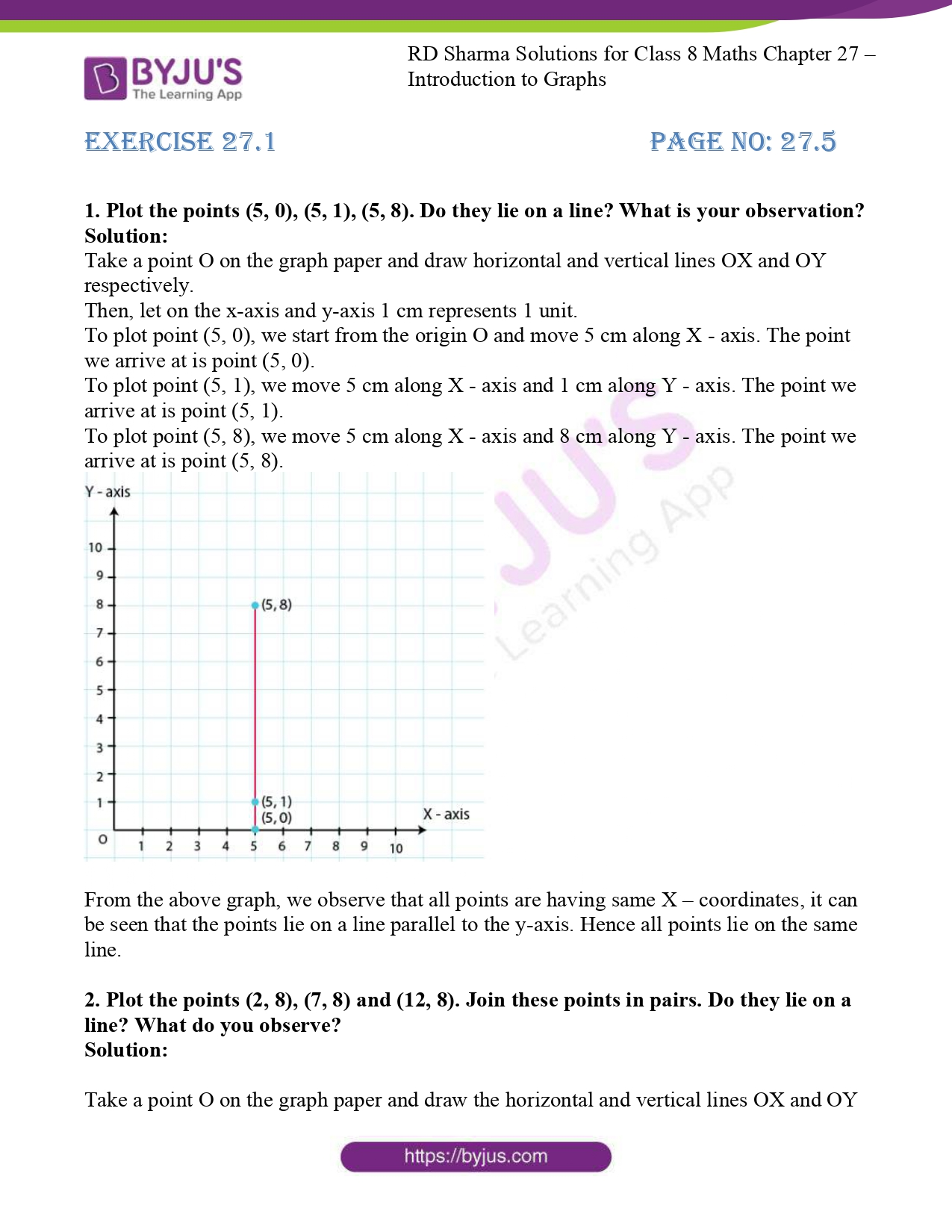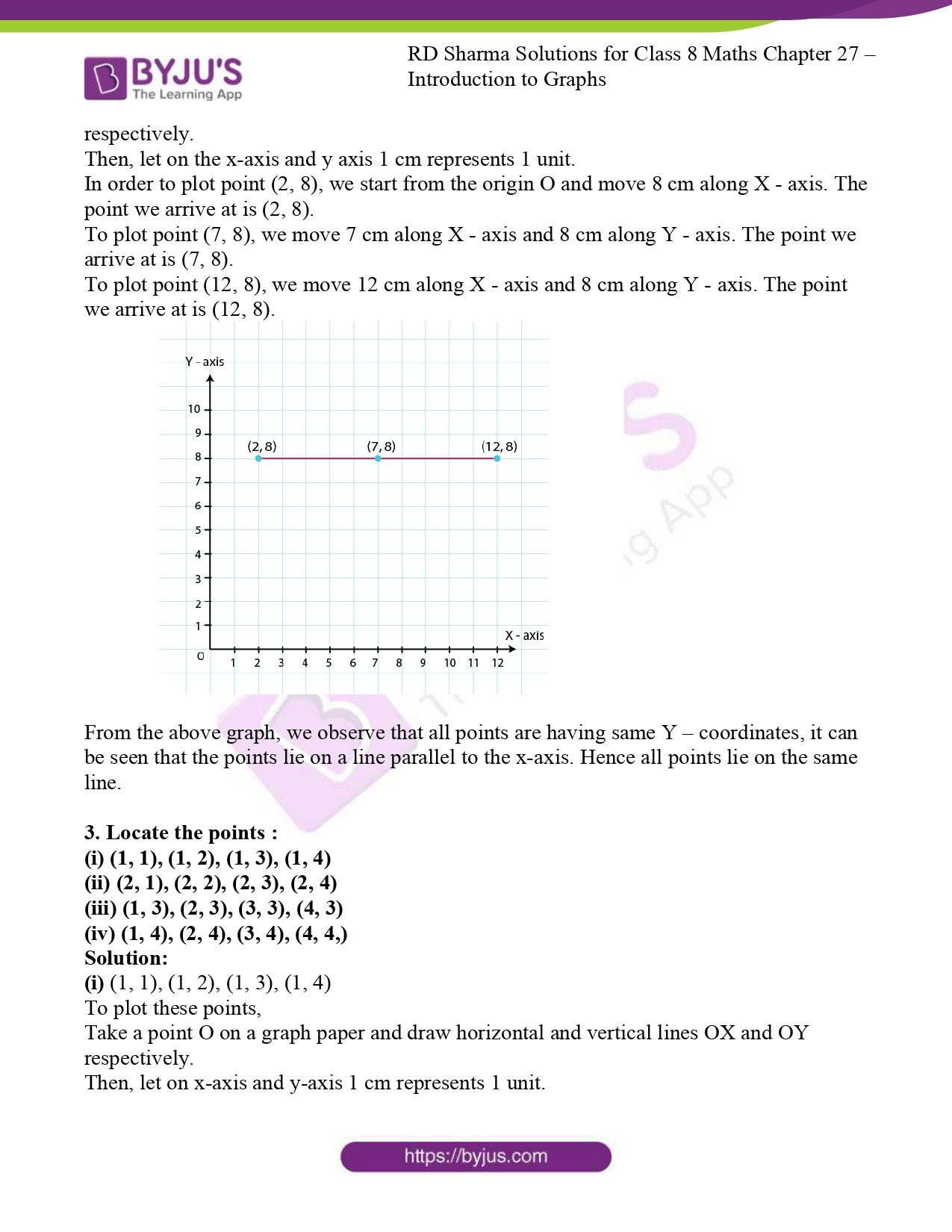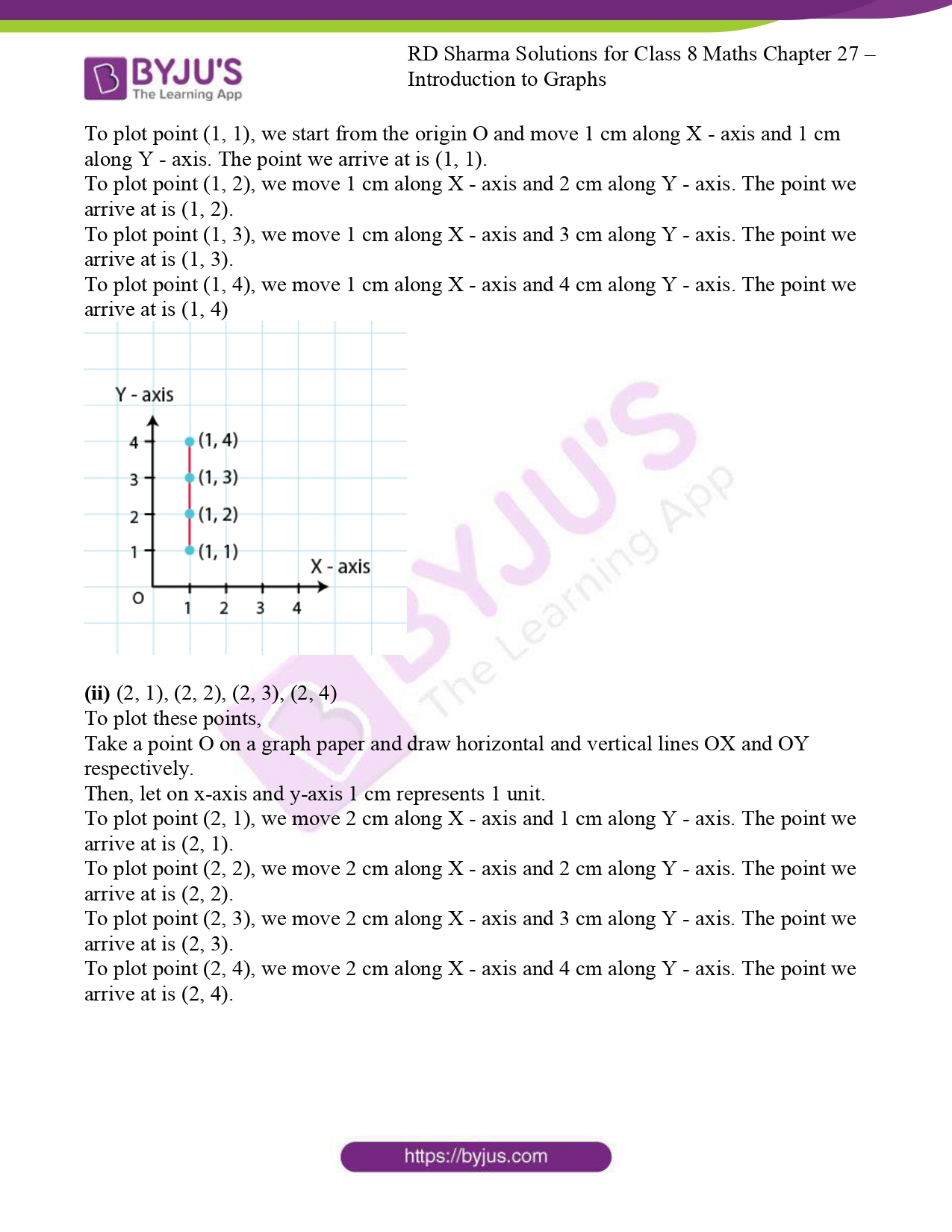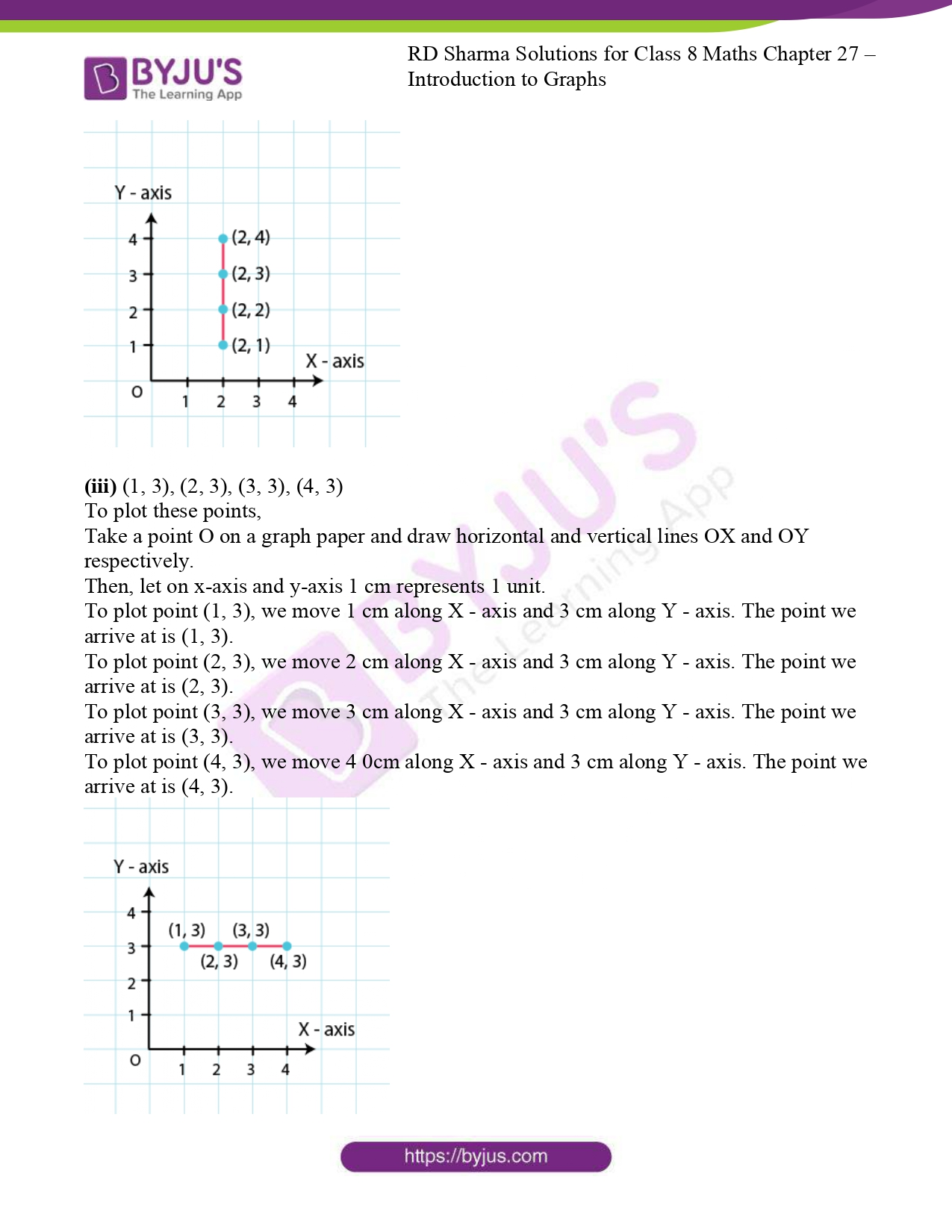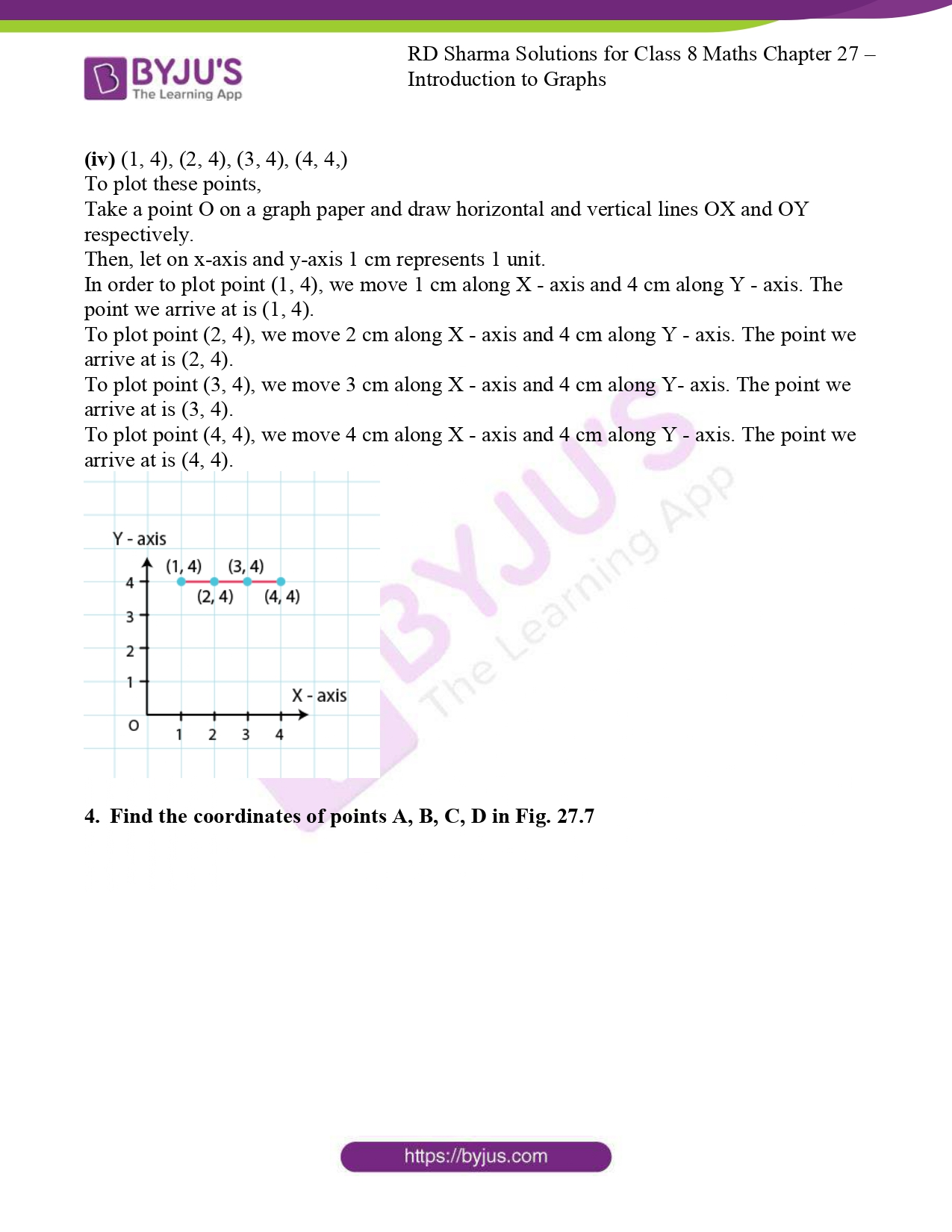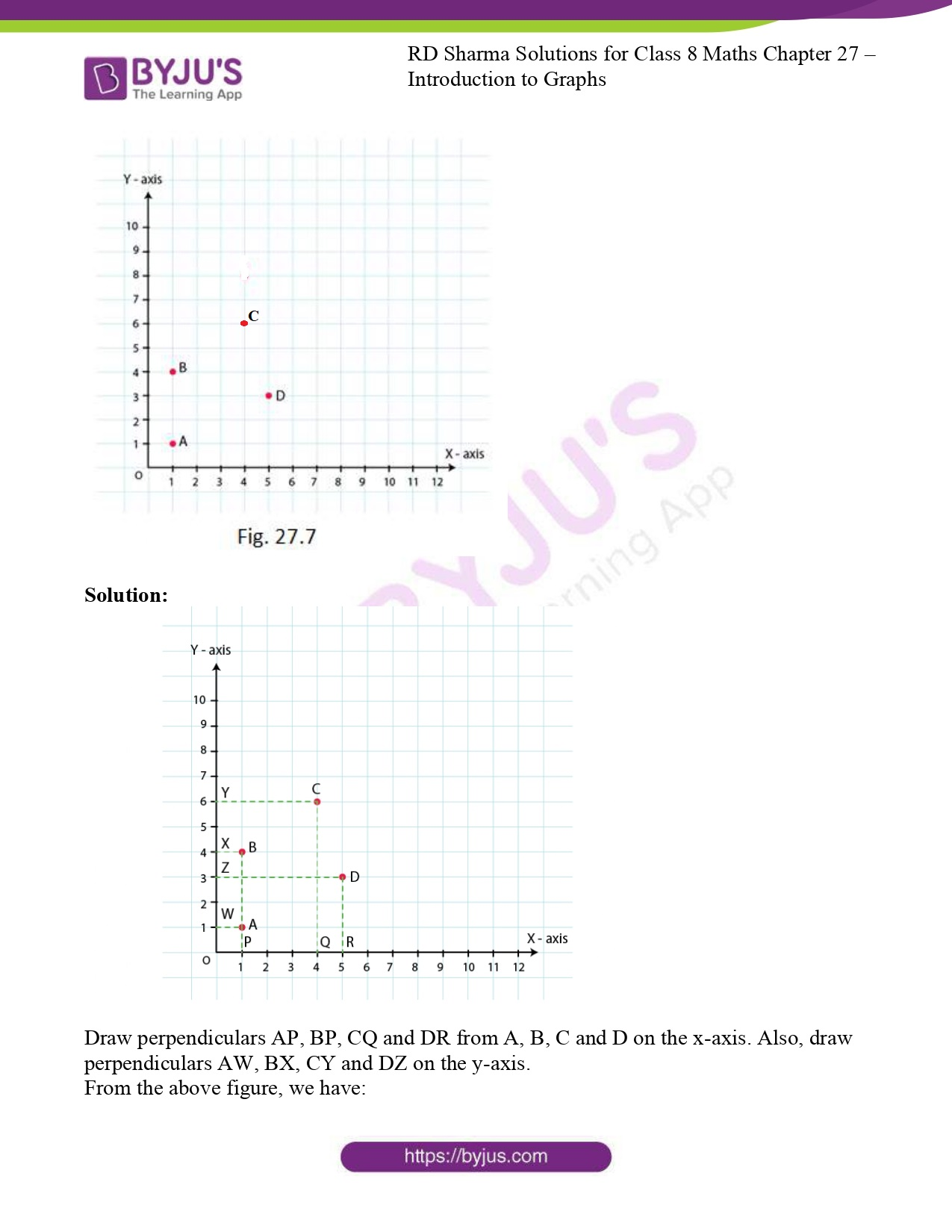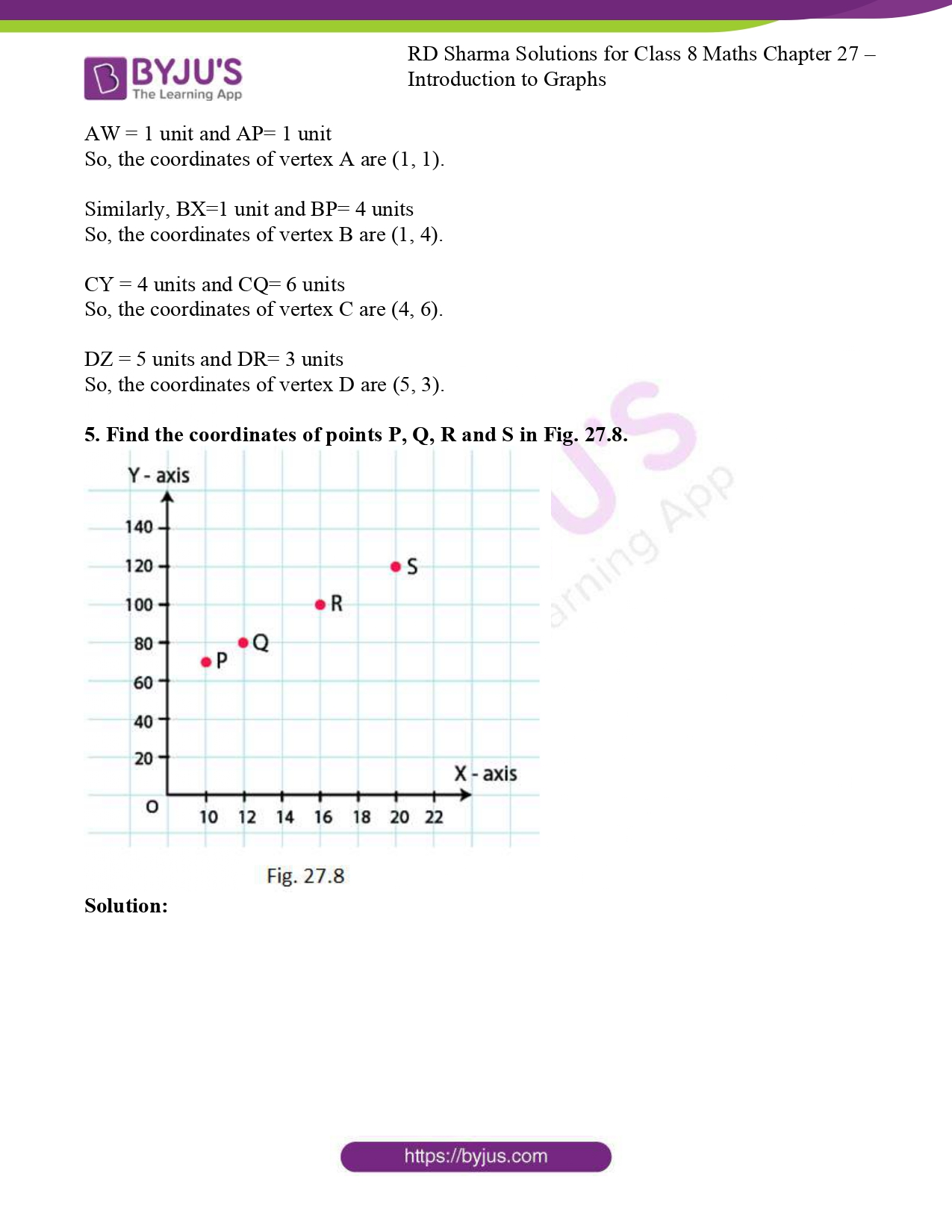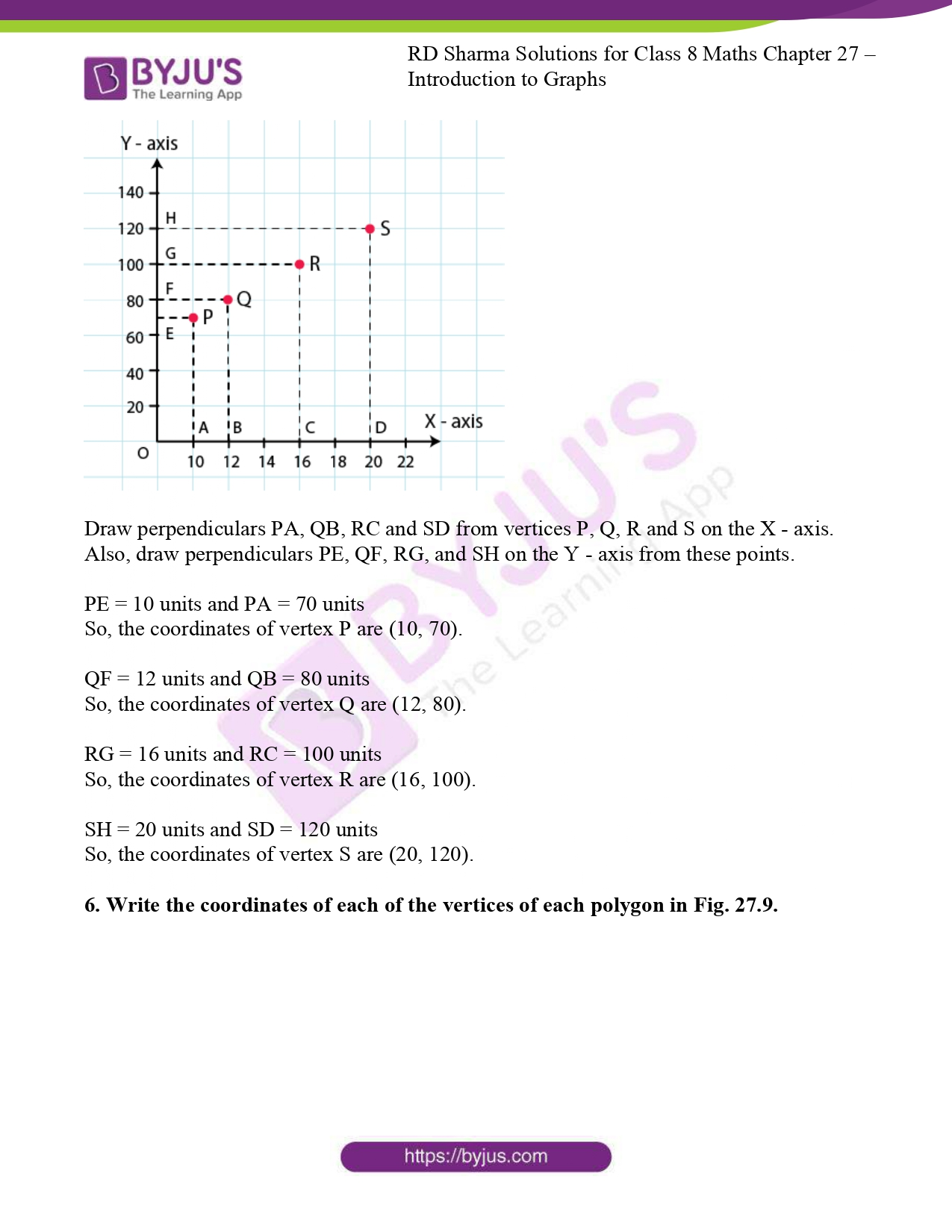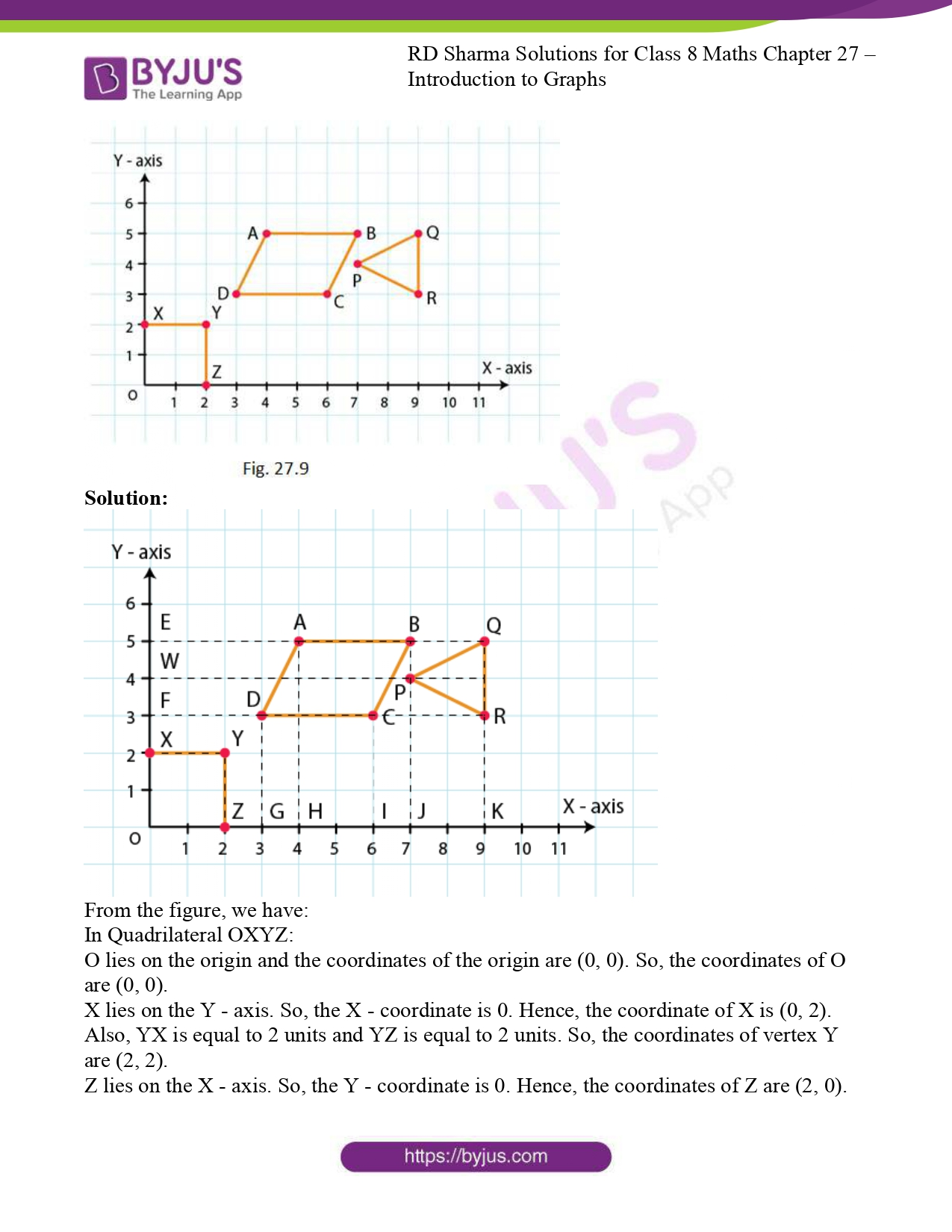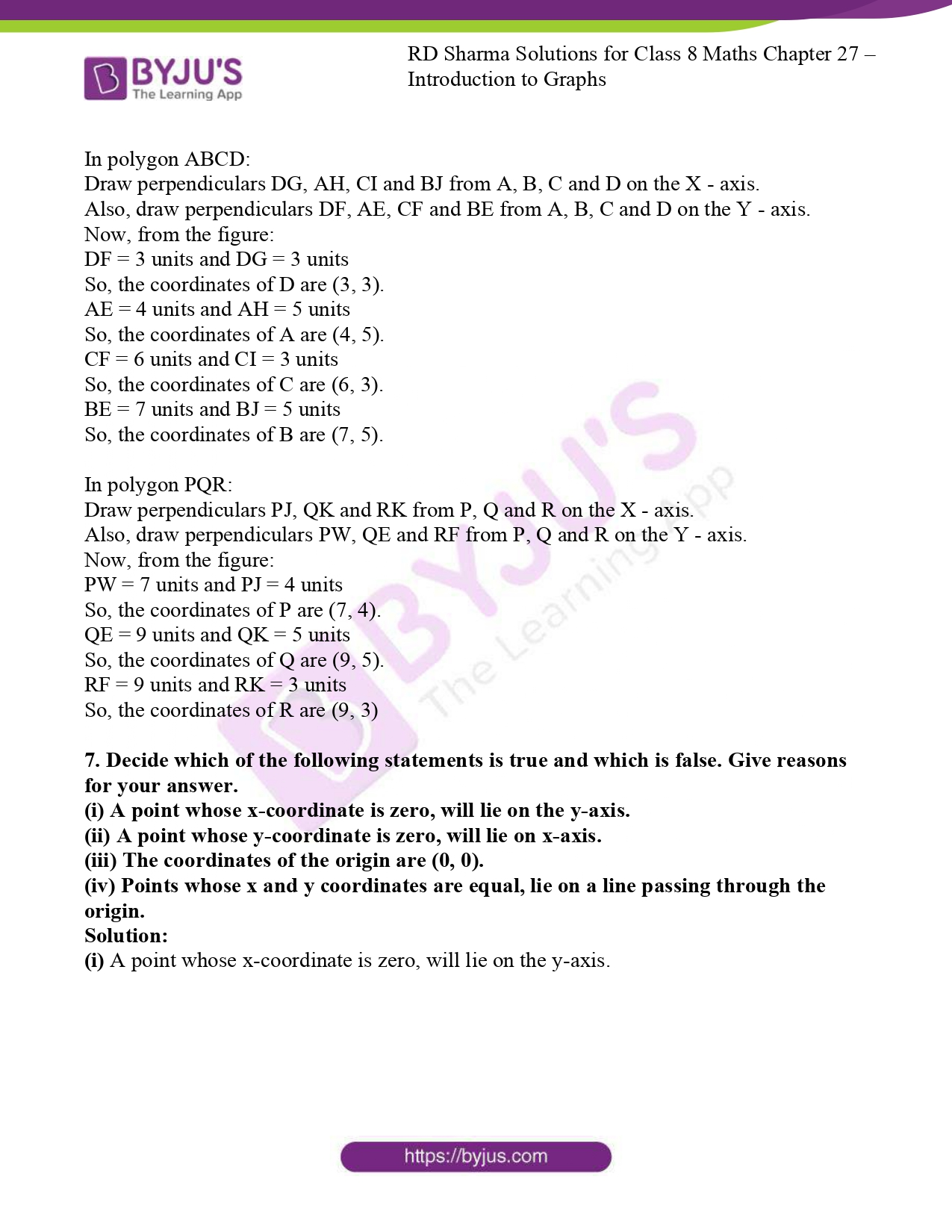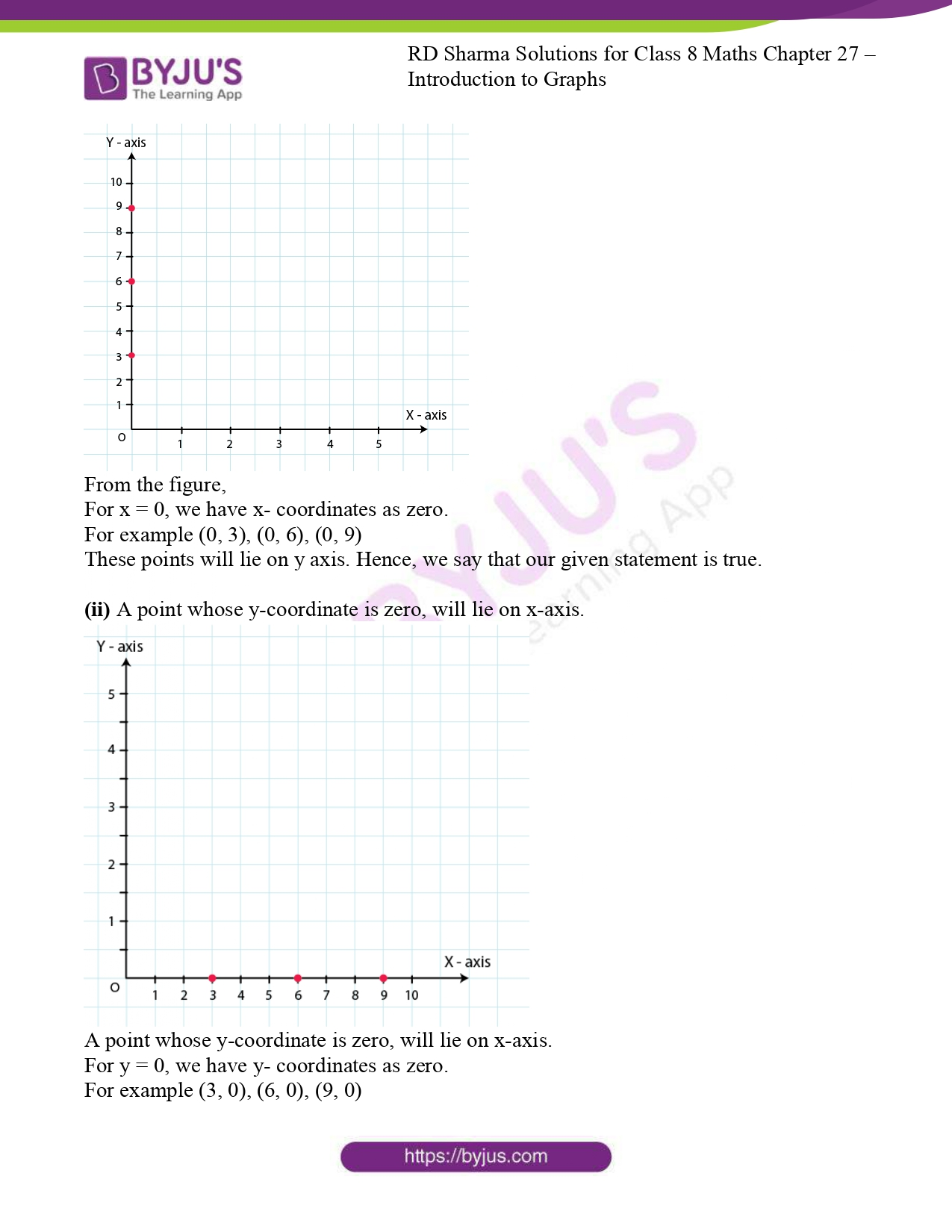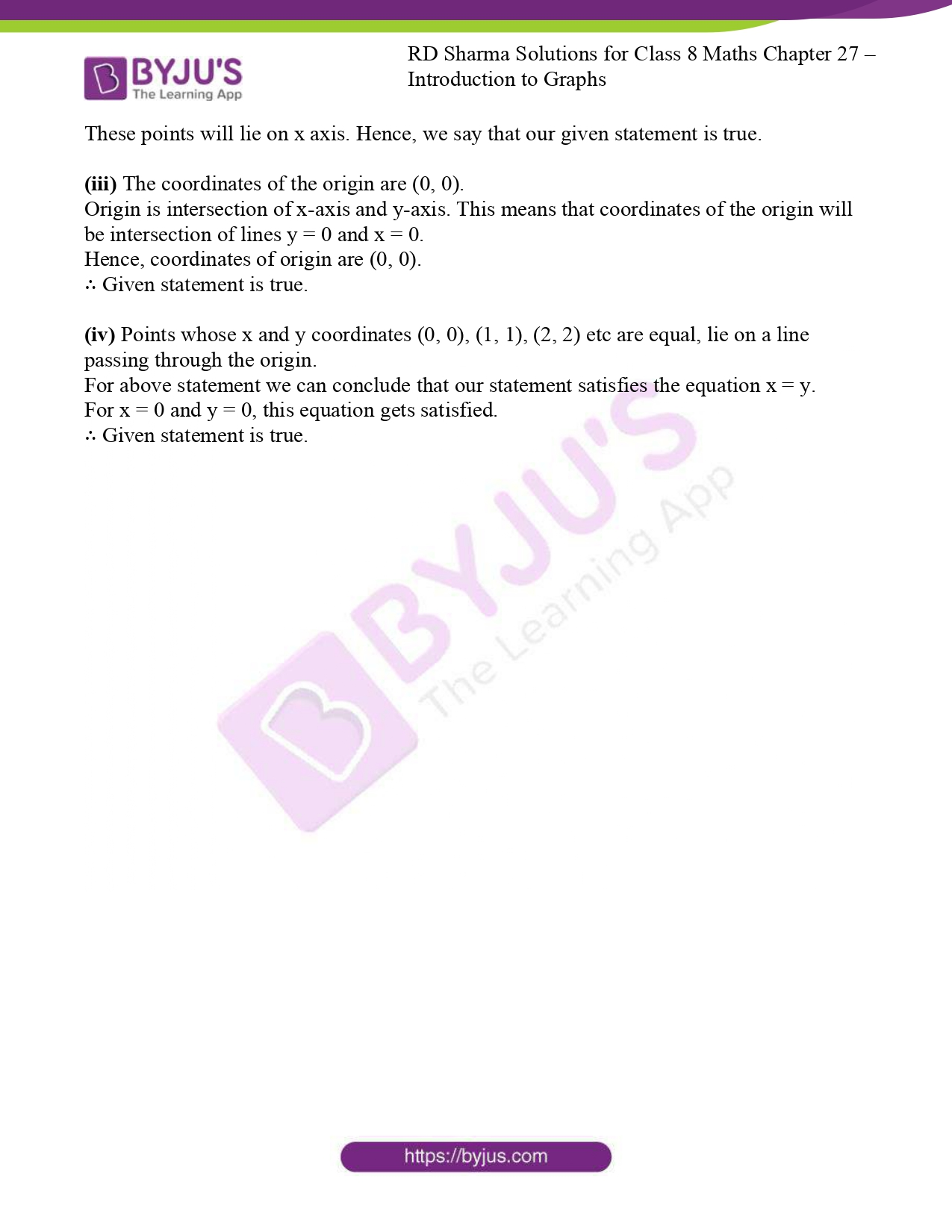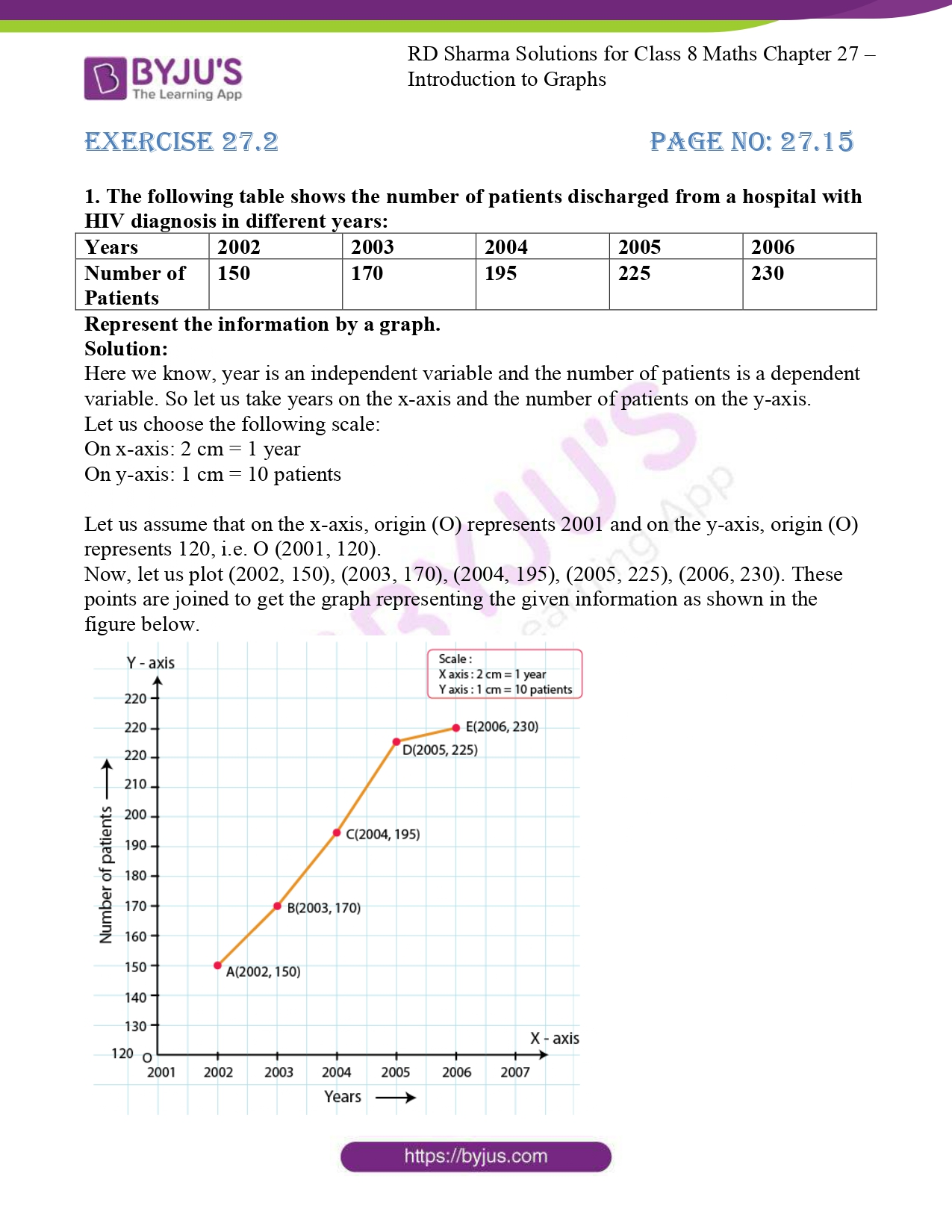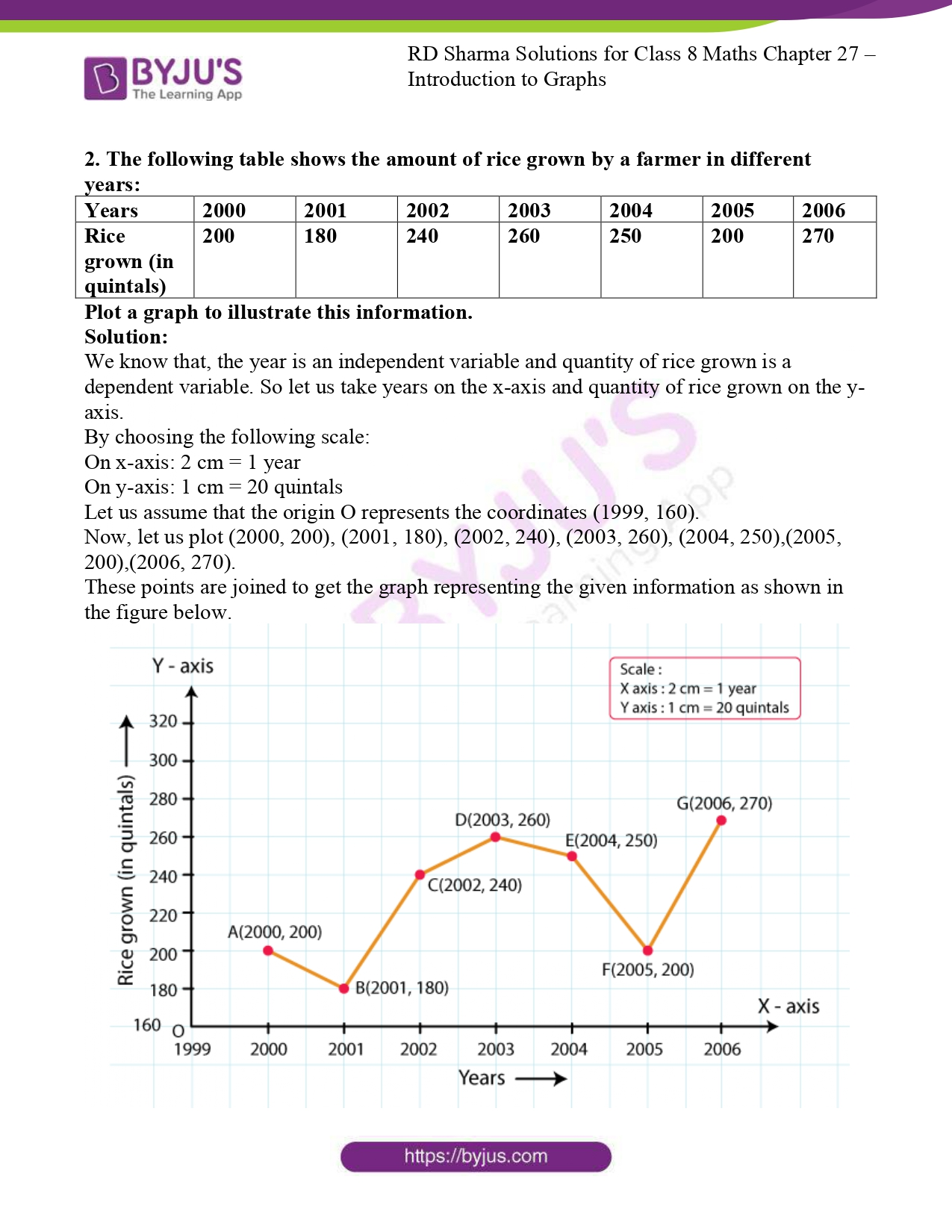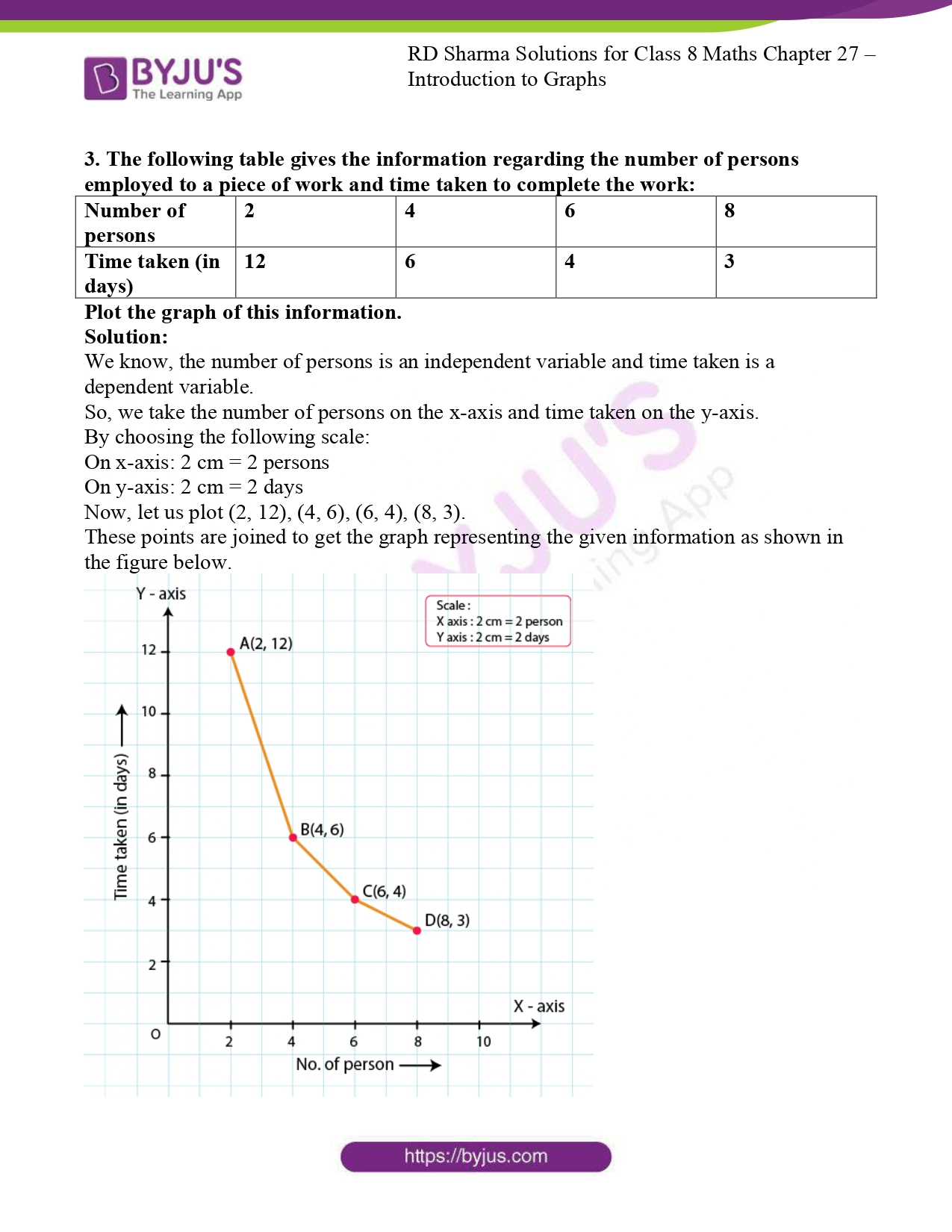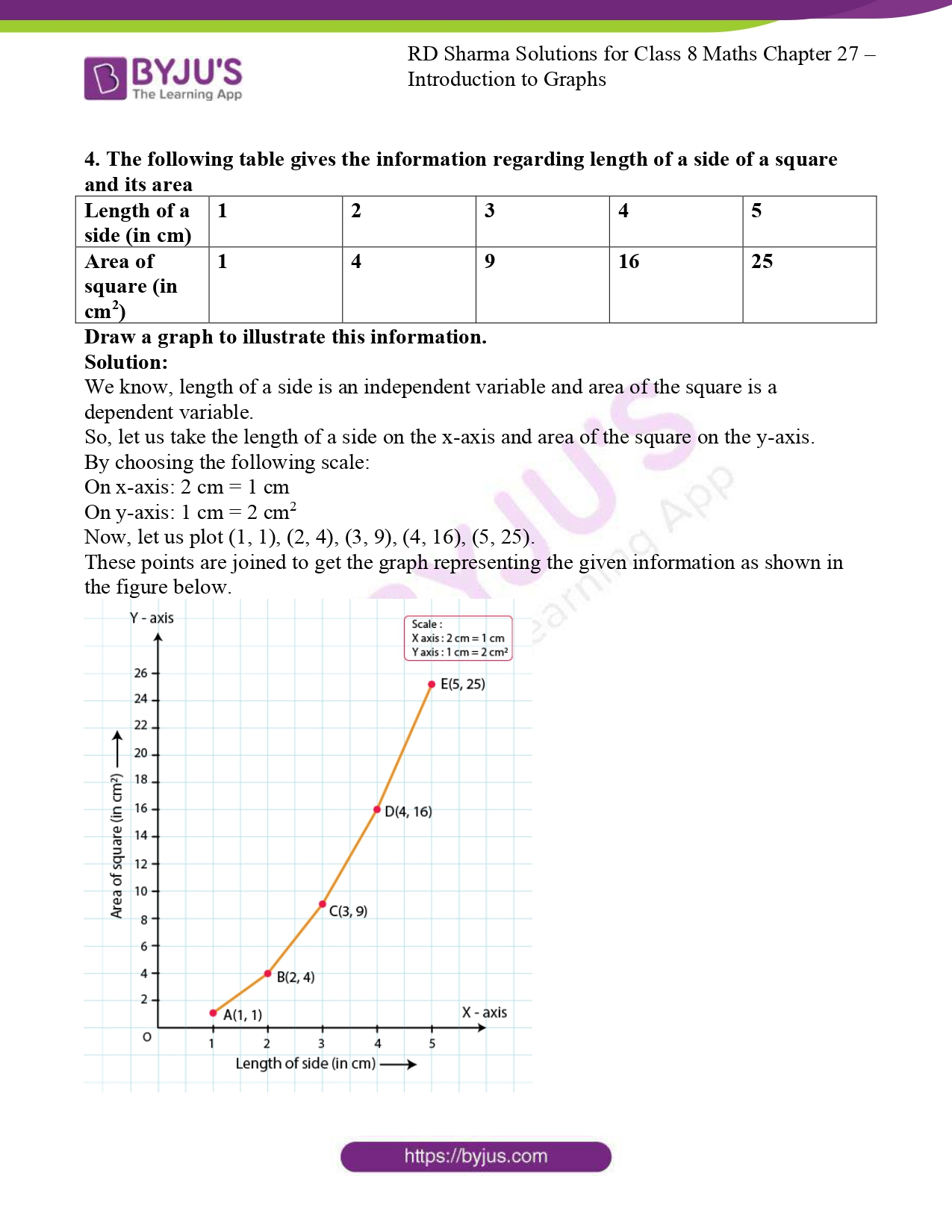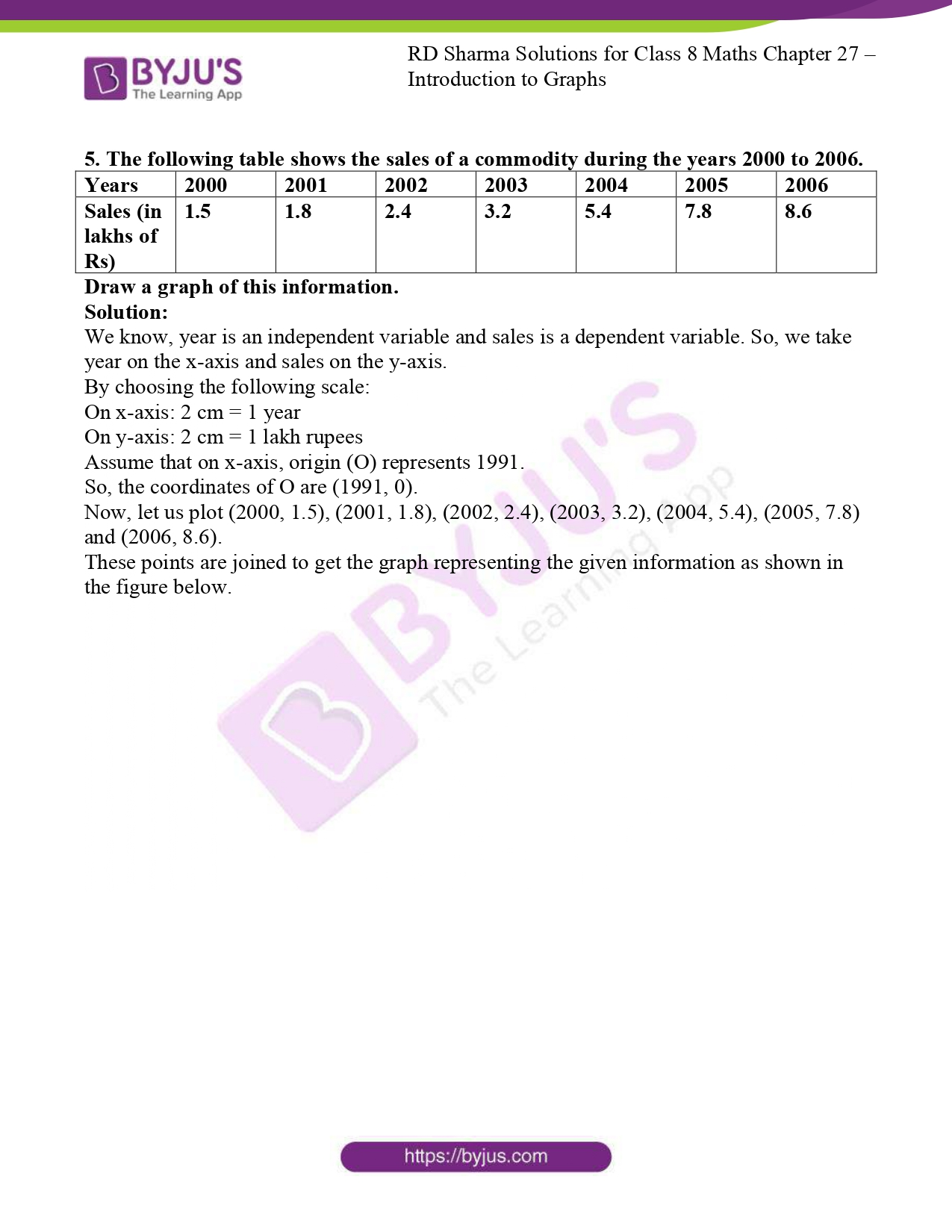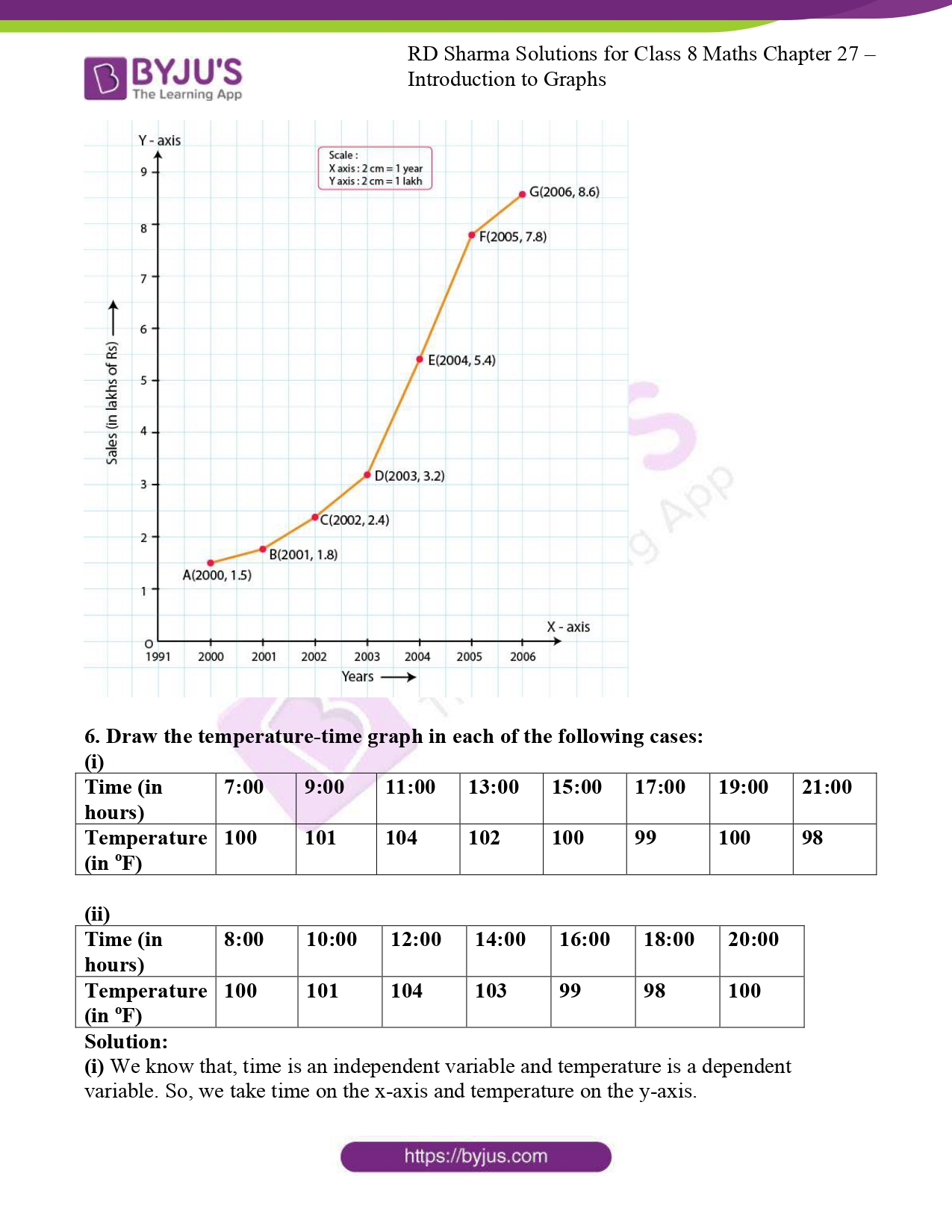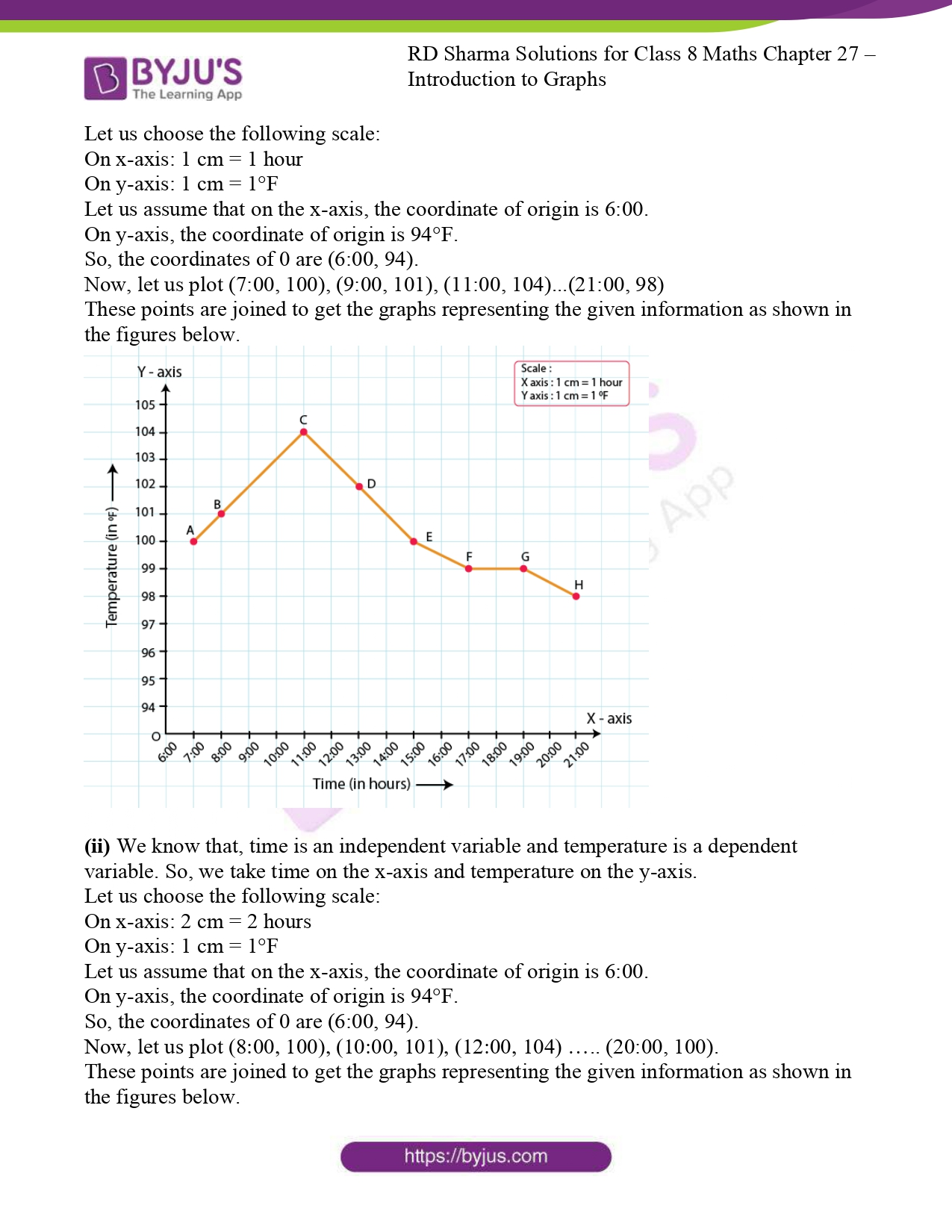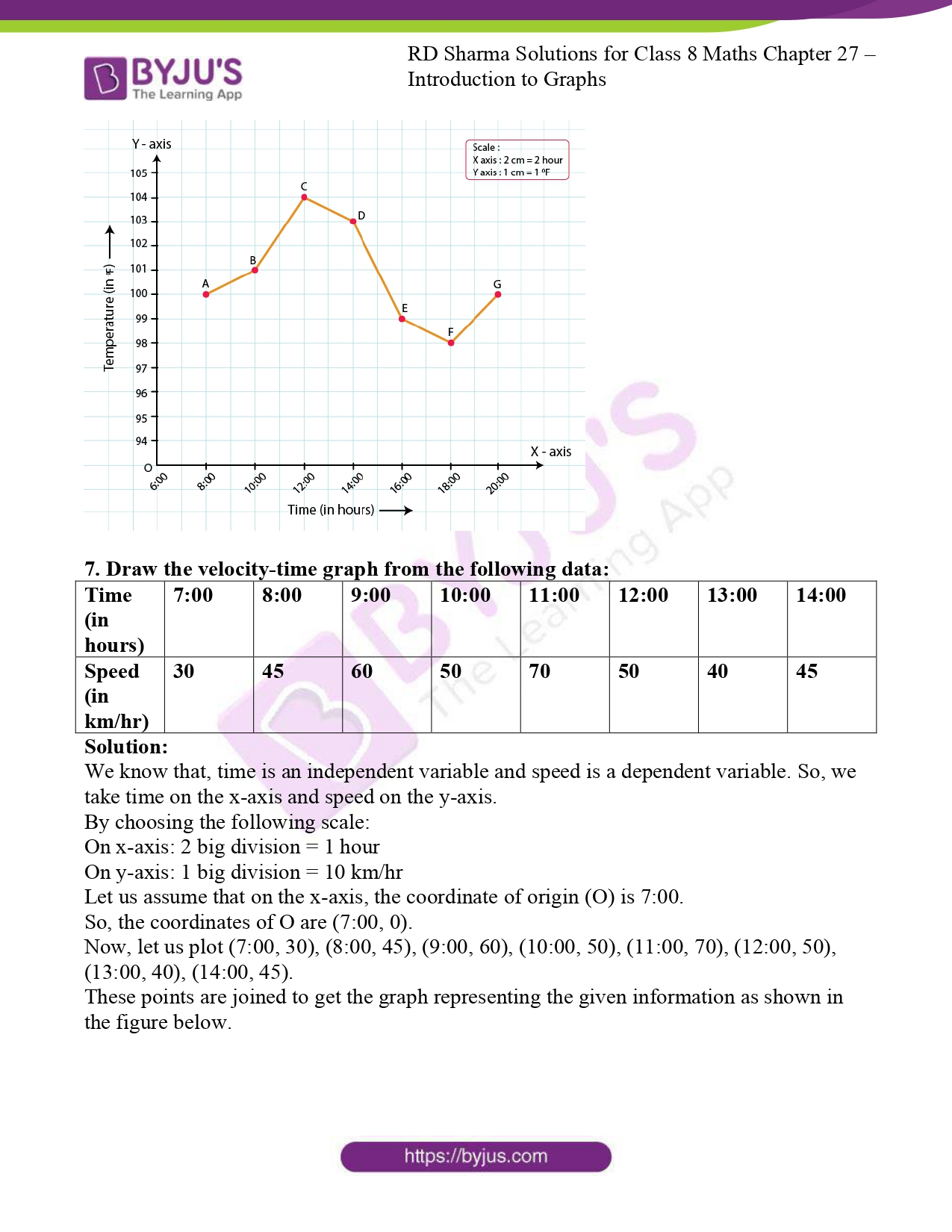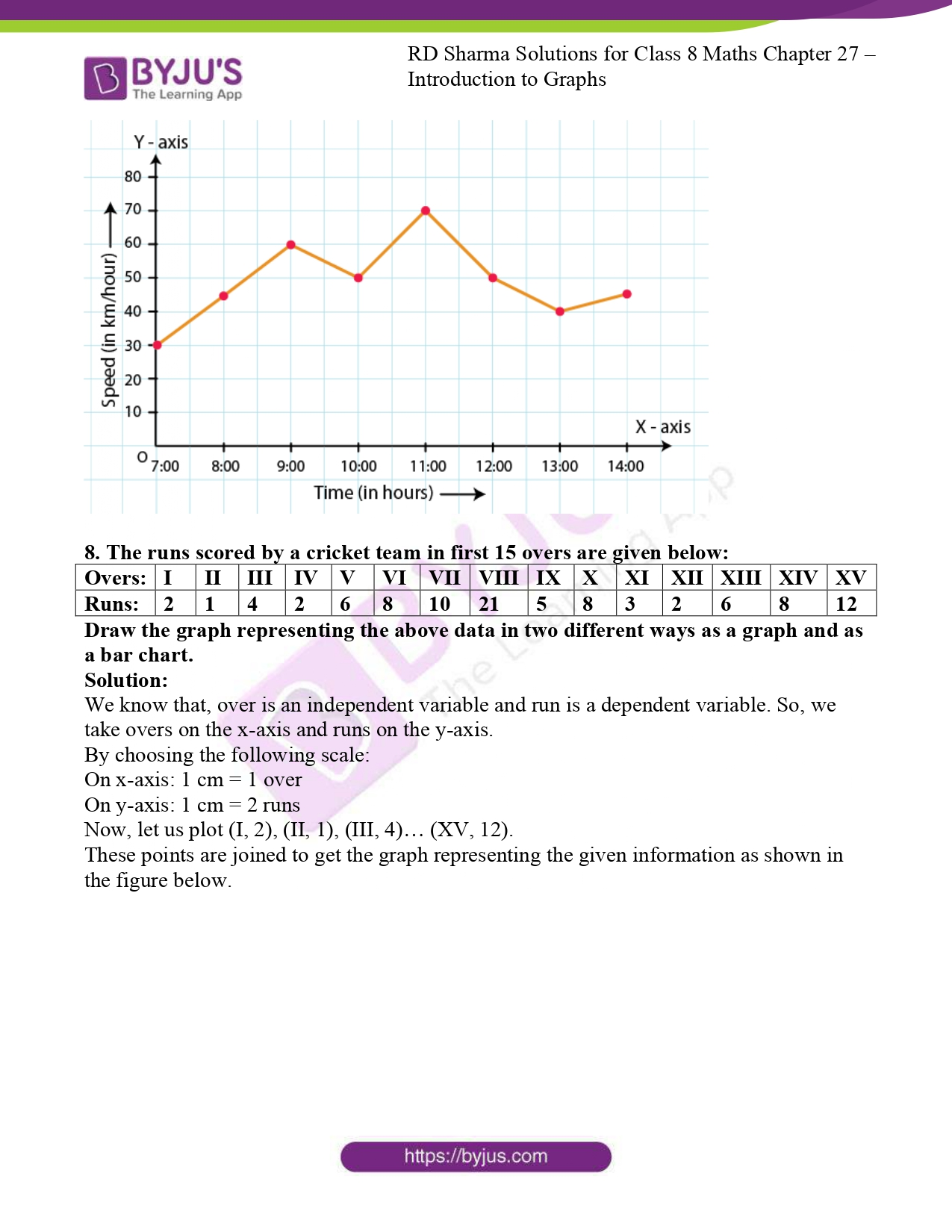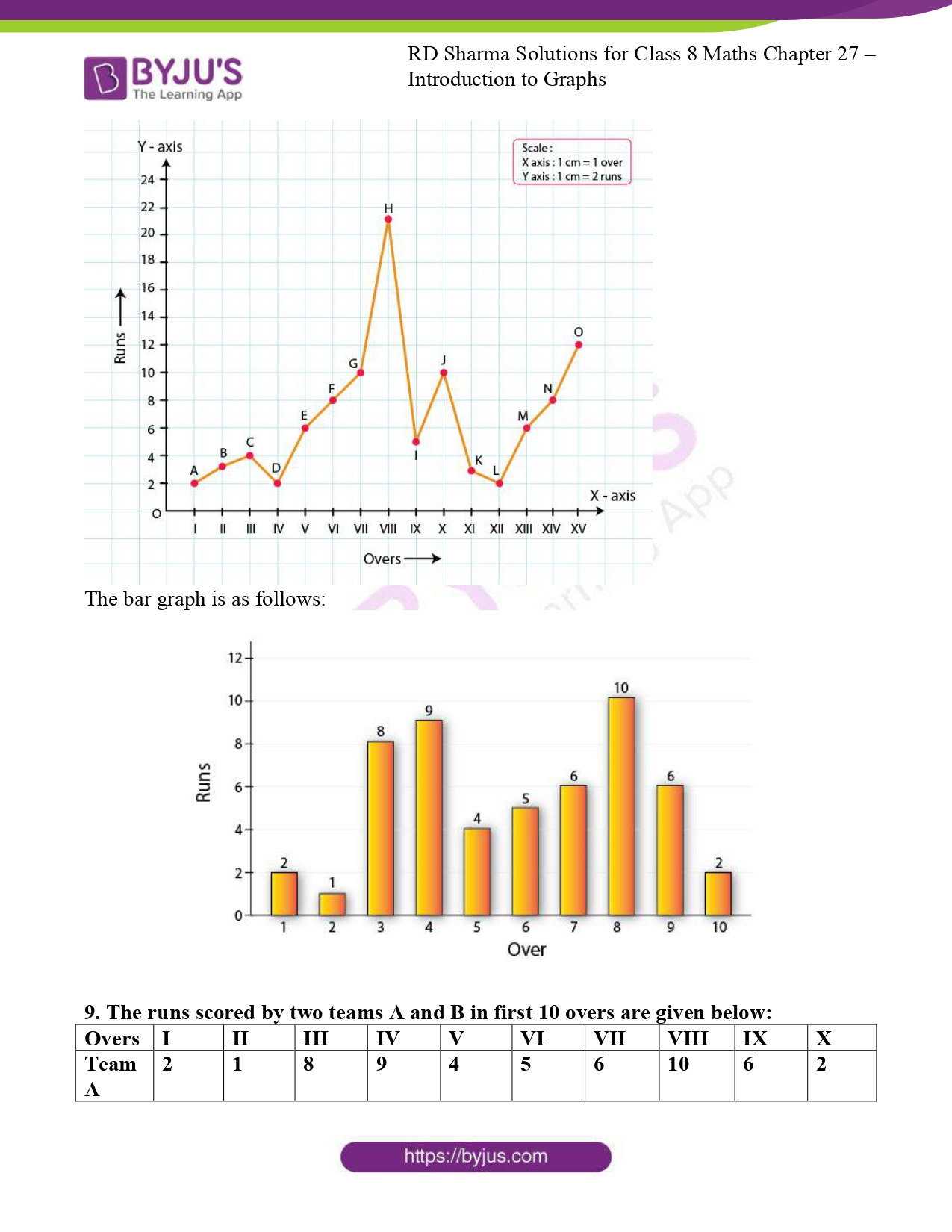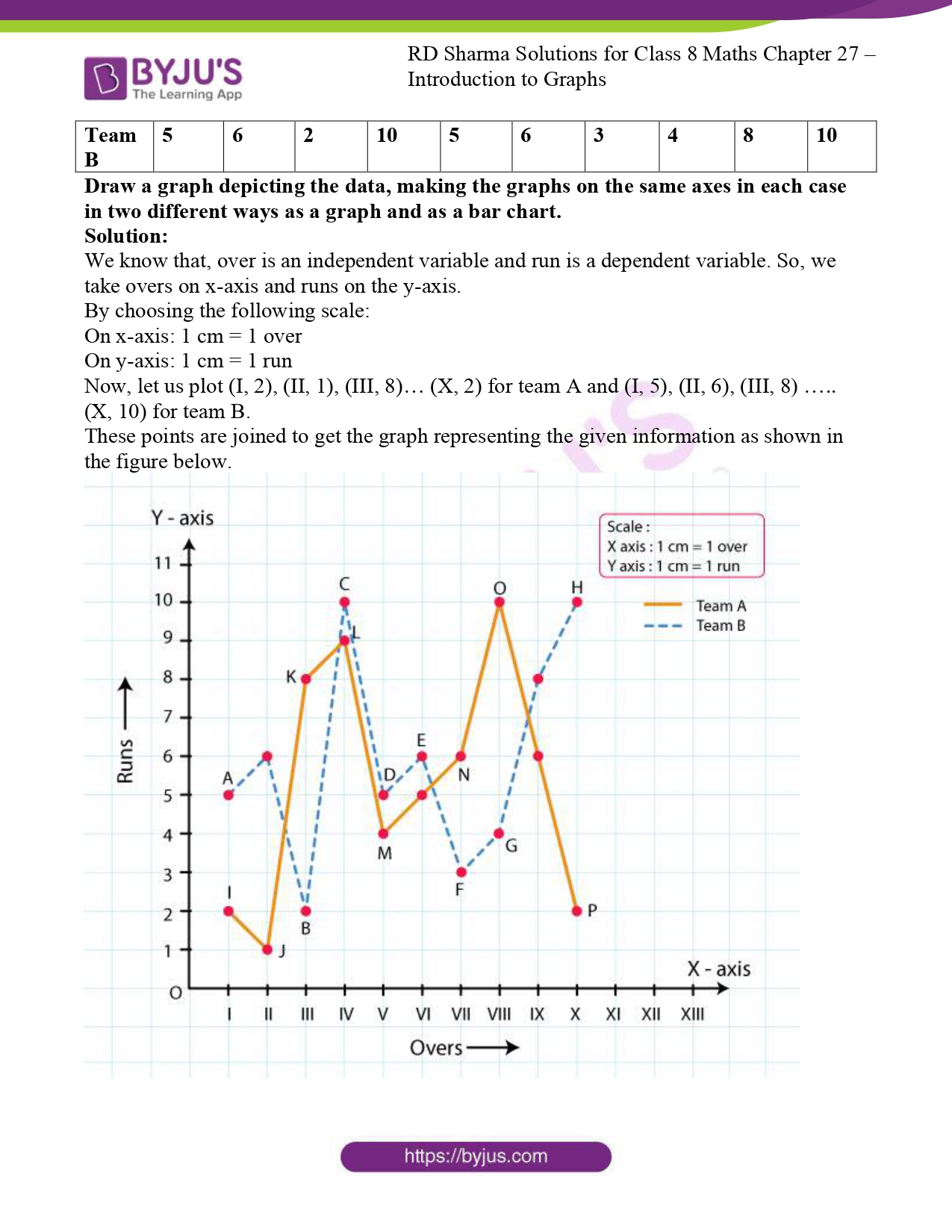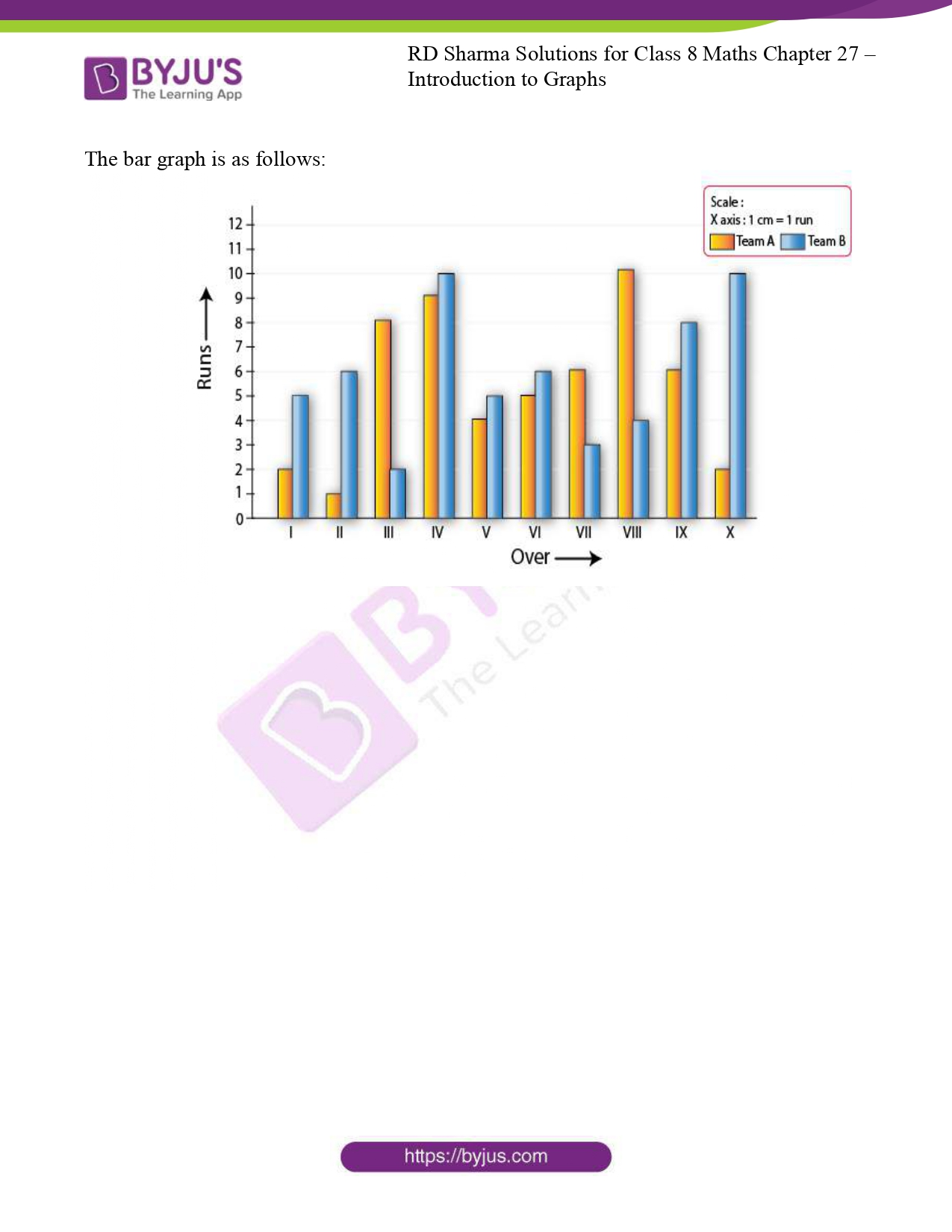### Access RD Sharma Solutions for Class 8 Maths Chapter 27 Introduction to Graphs

EXERCISE 27.1 PAGE NO: 27.5

1. Plot the points (5, 0), (5, 1), (5, 8). Do they lie on a line? What is your observation?

Solution:

Take a point O on the graph paper and draw horizontal and vertical lines OX and OY respectively.

Then, let on the x-axis and y-axis 1 cm represents 1 unit.

To plot point (5, 0), we start from the origin O and move 5 cm along X – axis. The point we arrive at is point (5, 0).

To plot point (5, 1), we move 5 cm along X – axis and 1 cm along Y – axis. The point we arrive at is point (5, 1).

To plot point (5, 8), we move 5 cm along X – axis and 8 cm along Y – axis. The point we arrive at is point (5, 8).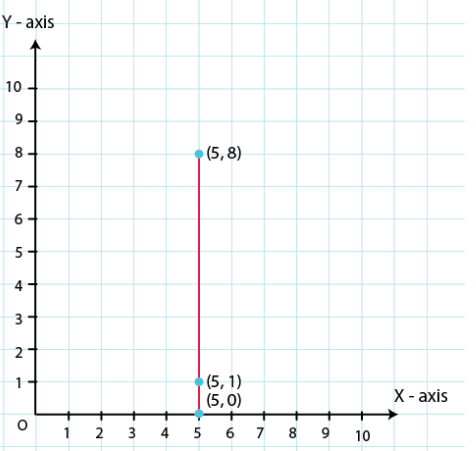From the above graph, we observe that all points are having same X – coordinates, it can be seen that the points lie on a line parallel to the y-axis. Hence all points lie on the same line.

2. Plot the points (2, 8), (7, 8) and (12, 8). Join these points in pairs. Do they lie on a line? What do you observe?

Solution:

Take a point O on the graph paper and draw the horizontal and vertical lines OX and OY respectively.

Then, let on the x-axis and y axis 1 cm represents 1 unit.

In order to plot point (2, 8), we start from the origin O and move 8 cm along X – axis. The point we arrive at is (2, 8).

To plot point (7, 8), we move 7 cm along X – axis and 8 cm along Y – axis. The point we arrive at is (7, 8).

To plot point (12, 8), we move 12 cm along X – axis and 8 cm along Y – axis. The point we arrive at is (12, 8).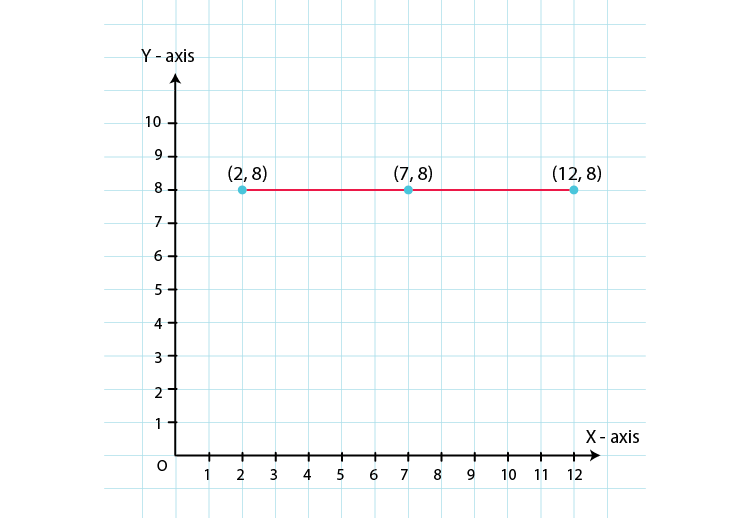From the above graph, we observe that all points are having same Y – coordinates, it can be seen that the points lie on a line parallel to the x-axis. Hence all points lie on the same line.

3. Locate the points :
(i) (1, 1), (1, 2), (1, 3), (1, 4)
(ii) (2, 1), (2, 2), (2, 3), (2, 4)
(iii) (1, 3), (2, 3), (3, 3), (4, 3)
(iv) (1, 4), (2, 4), (3, 4), (4, 4,)

Solution:

(i) (1, 1), (1, 2), (1, 3), (1, 4)

To plot these points,

Take a point O on a graph paper and draw horizontal and vertical lines OX and OY respectively.

Then, let on x-axis and y-axis 1 cm represents 1 unit.

To plot point (1, 1), we start from the origin O and move 1 cm along X – axis and 1 cm along Y – axis. The point we arrive at is (1, 1).

To plot point (1, 2), we move 1 cm along X – axis and 2 cm along Y – axis. The point we arrive at is (1, 2).

To plot point (1, 3), we move 1 cm along X – axis and 3 cm along Y – axis. The point we arrive at is (1, 3).

To plot point (1, 4), we move 1 cm along X – axis and 4 cm along Y – axis. The point we arrive at is (1, 4)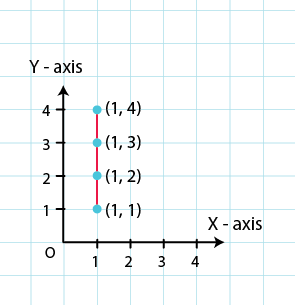(ii) (2, 1), (2, 2), (2, 3), (2, 4)

To plot these points,

Take a point O on a graph paper and draw horizontal and vertical lines OX and OY respectively.

Then, let on x-axis and y-axis 1 cm represents 1 unit.

To plot point (2, 1), we move 2 cm along X – axis and 1 cm along Y – axis. The point we arrive at is (2, 1).

To plot point (2, 2), we move 2 cm along X – axis and 2 cm along Y – axis. The point we arrive at is (2, 2).

To plot point (2, 3), we move 2 cm along X – axis and 3 cm along Y – axis. The point we arrive at is (2, 3).

To plot point (2, 4), we move 2 cm along X – axis and 4 cm along Y – axis. The point we arrive at is (2, 4).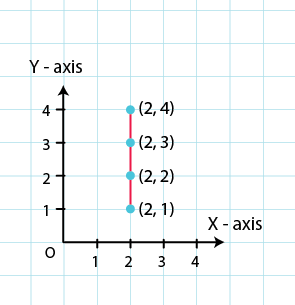(iii) (1, 3), (2, 3), (3, 3), (4, 3)

To plot these points,

Take a point O on a graph paper and draw horizontal and vertical lines OX and OY respectively.

Then, let on x-axis and y-axis 1 cm represents 1 unit.

To plot point (1, 3), we move 1 cm along X – axis and 3 cm along Y – axis. The point we arrive at is (1, 3).

To plot point (2, 3), we move 2 cm along X – axis and 3 cm along Y – axis. The point we arrive at is (2, 3).

To plot point (3, 3), we move 3 cm along X – axis and 3 cm along Y – axis. The point we arrive at is (3, 3).

To plot point (4, 3), we move 4 0cm along X – axis and 3 cm along Y – axis. The point we arrive at is (4, 3).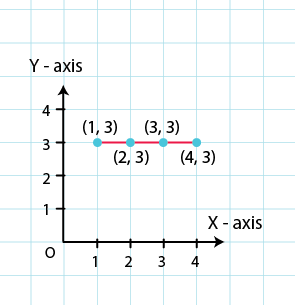(iv) (1, 4), (2, 4), (3, 4), (4, 4,)

To plot these points,

Take a point O on a graph paper and draw horizontal and vertical lines OX and OY respectively.

Then, let on x-axis and y-axis 1 cm represents 1 unit.

In order to plot point (1, 4), we move 1 cm along X – axis and 4 cm along Y – axis. The point we arrive at is (1, 4).

To plot point (2, 4), we move 2 cm along X – axis and 4 cm along Y – axis. The point we arrive at is (2, 4).

To plot point (3, 4), we move 3 cm along X – axis and 4 cm along Y- axis. The point we arrive at is (3, 4).

To plot point (4, 4), we move 4 cm along X – axis and 4 cm along Y – axis. The point we arrive at is (4, 4).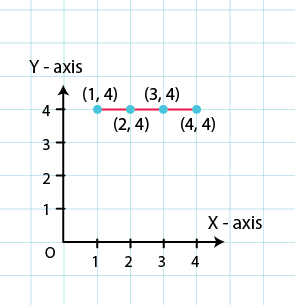4. Find the coordinates of points A, B, C, D in Fig. 27.7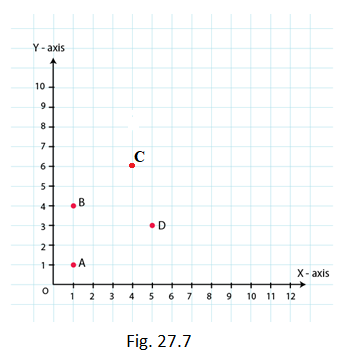Solution: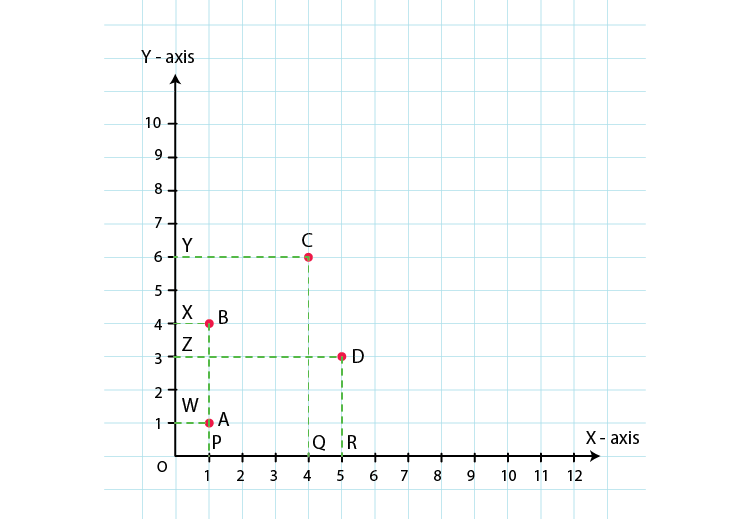Draw perpendiculars AP, BP, CQ and DR from A, B, C and D on the x-axis. Also, draw perpendiculars AW, BX, CY and DZ on the y-axis.

From the above figure, we have:

AW = 1 unit and AP= 1 unit

So, the coordinates of vertex A are (1, 1).

Similarly, BX=1 unit and BP= 4 units

So, the coordinates of vertex B are (1, 4).

CY = 4 units and CQ= 6 units

So, the coordinates of vertex C are (4, 6).

DZ = 5 units and DR= 3 units

So, the coordinates of vertex D are (5, 3).

5. Find the coordinates of points P, Q, R and S in Fig. 27.8.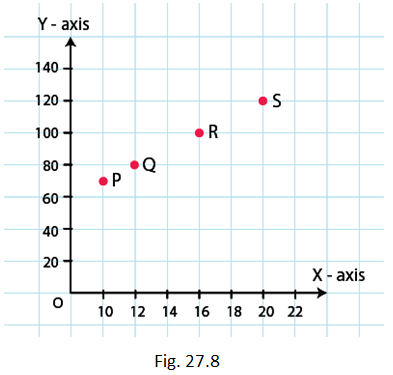Solution: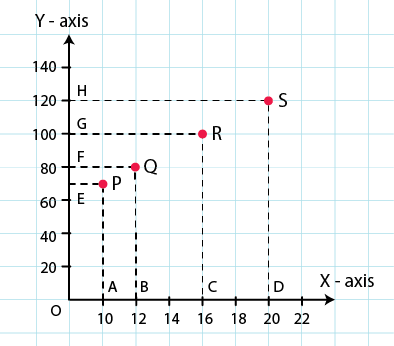Draw perpendiculars PA, QB, RC and SD from vertices P, Q, R and S on the X – axis. Also, draw perpendiculars PE, QF, RG, and SH on the Y – axis from these points.

PE = 10 units and PA = 70 units

So, the coordinates of vertex P are (10, 70).

QF = 12 units and QB = 80 units

So, the coordinates of vertex Q are (12, 80).

RG = 16 units and RC = 100 units

So, the coordinates of vertex R are (16, 100).

SH = 20 units and SD = 120 units

So, the coordinates of vertex S are (20, 120).

6. Write the coordinates of each of the vertices of each polygon in Fig. 27.9.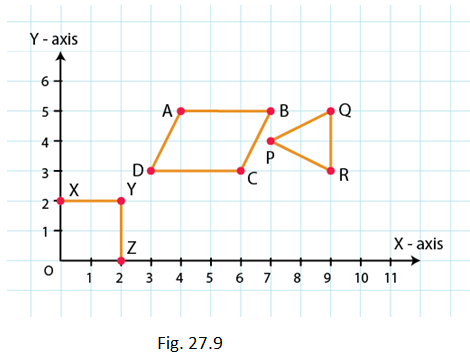Solution: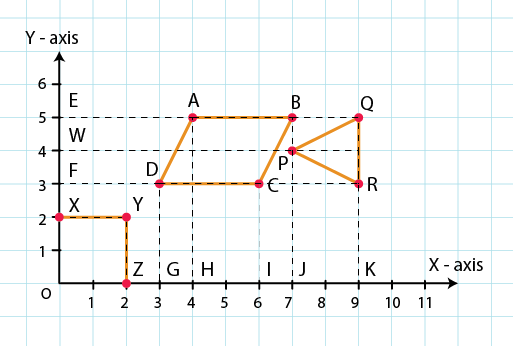From the figure, we have:

O lies on the origin and the coordinates of the origin are (0, 0). So, the coordinates of O are (0, 0).

X lies on the Y – axis. So, the X – coordinate is 0. Hence, the coordinate of X is (0, 2).

Also, YX is equal to 2 units and YZ is equal to 2 units. So, the coordinates of vertex Y are (2, 2).

Z lies on the X – axis. So, the Y – coordinate is 0. Hence, the coordinates of Z are (2, 0).

In polygon ABCD:

Draw perpendiculars DG, AH, CI and BJ from A, B, C and D on the X – axis.

Also, draw perpendiculars DF, AE, CF and BE from A, B, C and D on the Y – axis.

Now, from the figure:

DF = 3 units and DG = 3 units

So, the coordinates of D are (3, 3).

AE = 4 units and AH = 5 units

So, the coordinates of A are (4, 5).

CF = 6 units and CI = 3 units

So, the coordinates of C are (6, 3).

BE = 7 units and BJ = 5 units

So, the coordinates of B are (7, 5).

In polygon PQR:

Draw perpendiculars PJ, QK and RK from P, Q and R on the X – axis.

Also, draw perpendiculars PW, QE and RF from P, Q and R on the Y – axis.

Now, from the figure:

PW = 7 units and PJ = 4 units

So, the coordinates of P are (7, 4).

QE = 9 units and QK = 5 units

So, the coordinates of Q are (9, 5).

RF = 9 units and RK = 3 units

So, the coordinates of R are (9, 3)

7. Decide which of the following statements is true and which is false. Give reasons for your answer.
(i) A point whose x-coordinate is zero, will lie on the y-axis.
(ii) A point whose y-coordinate is zero, will lie on x-axis.
(iii) The coordinates of the origin are (0, 0).
(iv) Points whose x and y coordinates are equal, lie on a line passing through the origin.

Solution:

(i) A point whose x-coordinate is zero, will lie on the y-axis.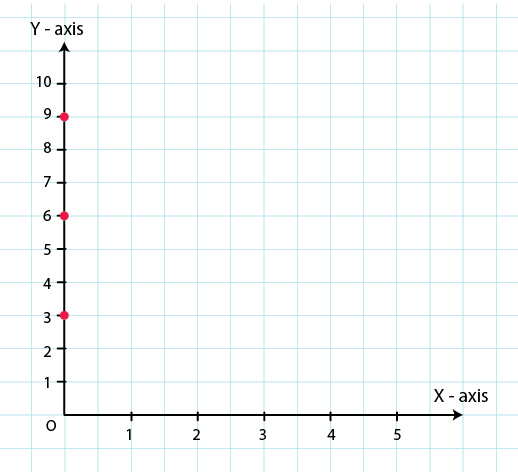From the figure,

For x = 0, we have x- coordinates as zero.

For example (0, 3), (0, 6), (0, 9)

These points will lie on y axis. Hence, we say that our given statement is true.

(ii) A point whose y-coordinate is zero, will lie on x-axis.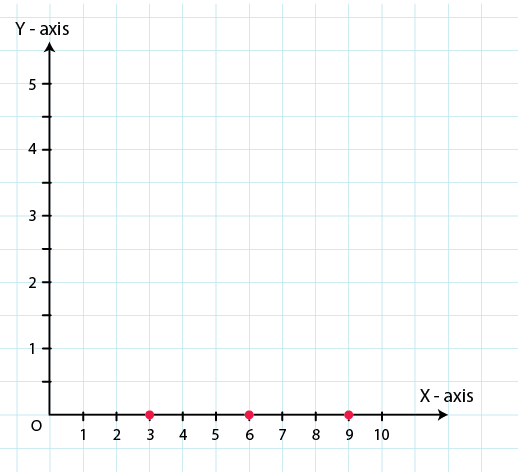A point whose y-coordinate is zero, will lie on x-axis.

For y = 0, we have y- coordinates as zero.

For example (3, 0), (6, 0), (9, 0)

These points will lie on x axis. Hence, we say that our given statement is true.

(iii) The coordinates of the origin are (0, 0).

Origin is intersection of x-axis and y-axis. This means that coordinates of the origin will be intersection of lines y = 0 and x = 0.

Hence, coordinates of origin are (0, 0).

∴ Given statement is true.

(iv) Points whose x and y coordinates (0, 0), (1, 1), (2, 2) etc are equal, lie on a line passing through the origin.

For above statement we can conclude that our statement satisfies the equation x = y.

For x = 0 and y = 0, this equation gets satisfied.

∴ Given statement is true.

EXERCISE 27.2 PAGE NO: 27.15

1. The following table shows the number of patients discharged from a hospital with HIV diagnosis in different years:

 Years 2002 2003 2004 2005 2006 Number of Patients 150 170 195 225 230

Represent the information by a graph.

Solution:

Here we know, year is an independent variable and the number of patients is a dependent variable. So let us take years on the x-axis and the number of patients on the y-axis.

Let us choose the following scale:

On x-axis: 2 cm = 1 year

On y-axis: 1 cm = 10 patients

Let us assume that on the x-axis, origin (O) represents 2001 and on the y-axis, origin (O) represents 120, i.e. O (2001, 120).

Now, let us plot (2002, 150), (2003, 170), (2004, 195), (2005, 225), (2006, 230). These points are joined to get the graph representing the given information as shown in the figure below.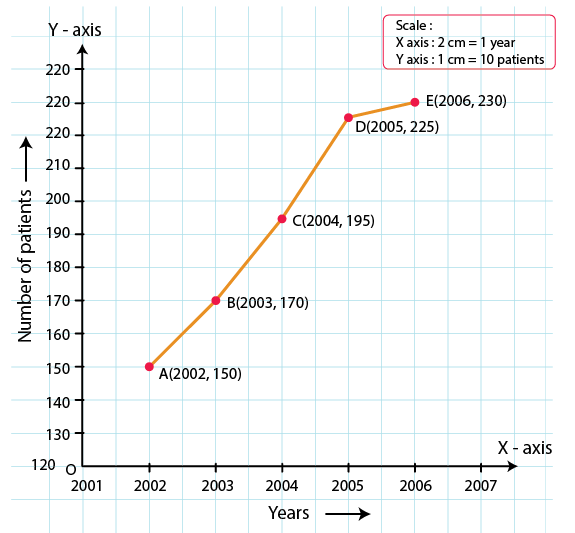2. The following table shows the amount of rice grown by a farmer in different years:

 Years 2000 2001 2002 2003 2004 2005 2006 Rice grown (in quintals) 200 180 240 260 250 200 270

Plot a graph to illustrate this information.

Solution:

We know that, the year is an independent variable and quantity of rice grown is a dependent variable. So let us take years on the x-axis and quantity of rice grown on the y-axis.

By choosing the following scale:

On x-axis: 2 cm = 1 year

On y-axis: 1 cm = 20 quintals

Let us assume that the origin O represents the coordinates (1999, 160).

Now, let us plot (2000, 200), (2001, 180), (2002, 240), (2003, 260), (2004, 250),(2005, 200),(2006, 270).

These points are joined to get the graph representing the given information as shown in the figure below.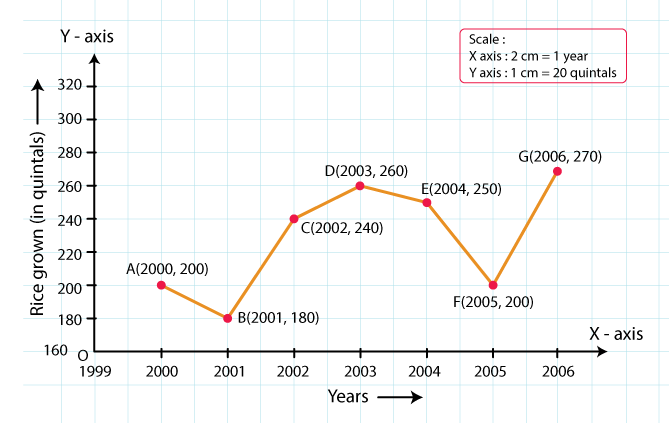3. The following table gives the information regarding the number of persons employed to a piece of work and time taken to complete the work:

 Number of persons 2 4 6 8 Time taken (in days) 12 6 4 3

Plot the graph of this information.

Solution:

We know, the number of persons is an independent variable and time taken is a dependent variable.

So, we take the number of persons on the x-axis and time taken on the y-axis.

By choosing the following scale:

On x-axis: 2 cm = 2 persons

On y-axis: 2 cm = 2 days

Now, let us plot (2, 12), (4, 6), (6, 4), (8, 3).

These points are joined to get the graph representing the given information as shown in the figure below.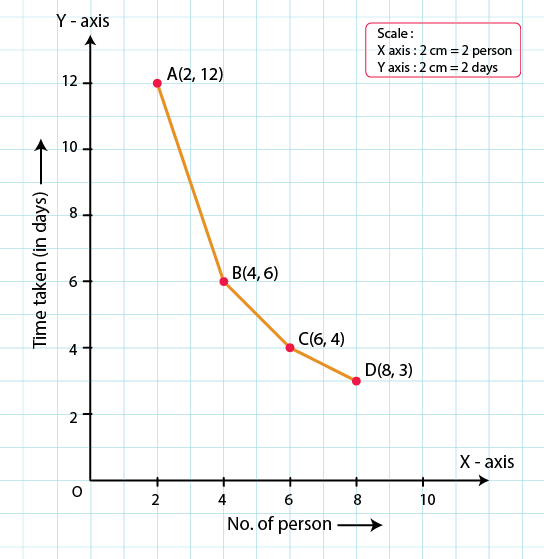4. The following table gives the information regarding length of a side of a square and its area

 Length of a side (in cm) 1 2 3 4 5 Area of square (in cm2) 1 4 9 16 25

Draw a graph to illustrate this information.

Solution:

We know, length of a side is an independent variable and area of the square is a dependent variable.

So, let us take the length of a side on the x-axis and area of the square on the y-axis.

By choosing the following scale:

On x-axis: 2 cm = 1 cm

On y-axis: 1 cm = 2 cm2

Now, let us plot (1, 1), (2, 4), (3, 9), (4, 16), (5, 25).

These points are joined to get the graph representing the given information as shown in the figure below.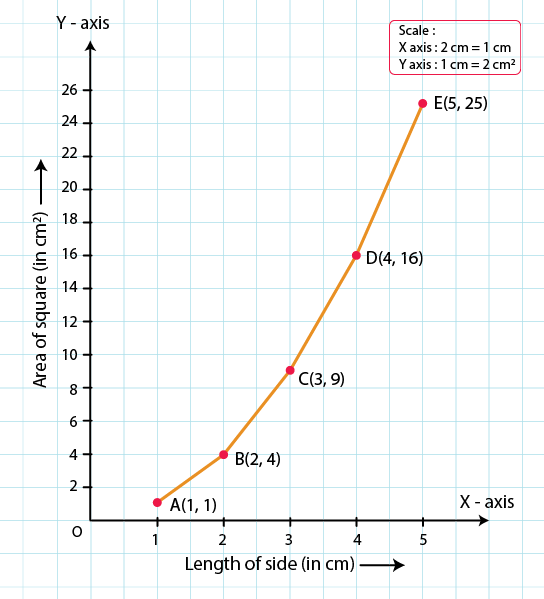5. The following table shows the sales of a commodity during the years 2000 to 2006.

 Years 2000 2001 2002 2003 2004 2005 2006 Sales (in lakhs of Rs) 1.5 1.8 2.4 3.2 5.4 7.8 8.6

Draw a graph of this information.

Solution:

We know, year is an independent variable and sales is a dependent variable. So, we take year on the x-axis and sales on the y-axis.

By choosing the following scale:

On x-axis: 2 cm = 1 year

On y-axis: 2 cm = 1 lakh rupees

Assume that on x-axis, origin (O) represents 1991.

So, the coordinates of O are (1991, 0).

Now, let us plot (2000, 1.5), (2001, 1.8), (2002, 2.4), (2003, 3.2), (2004, 5.4), (2005, 7.8) and (2006, 8.6).

These points are joined to get the graph representing the given information as shown in the figure below.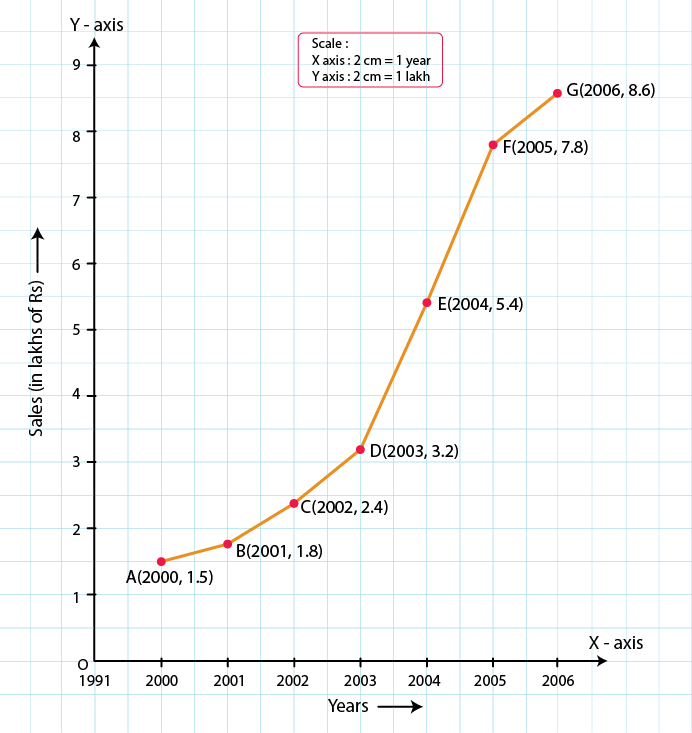6. Draw the temperature-time graph in each of the following cases:

(i)

 Time (in hours) 7:00 9:00 11:00 13:00 15:00 17:00 19:00 21:00 Temperature (in oF) 100 101 104 102 100 99 100 98

(ii)

 Time (in hours) 8:00 10:00 12:00 14:00 16:00 18:00 20:00 Temperature (in oF) 100 101 104 103 99 98 100

Solution:

(i) We know that, time is an independent variable and temperature is a dependent variable. So, we take time on the x-axis and temperature on the y-axis.

Let us choose the following scale:

On x-axis: 1 cm = 1 hour

On y-axis: 1 cm = 1°F

Let us assume that on the x-axis, the coordinate of origin is 6:00.

On y-axis, the coordinate of origin is 94°F.

So, the coordinates of 0 are (6:00, 94).

Now, let us plot (7:00, 100), (9:00, 101), (11:00, 104)…(21:00, 98)

These points are joined to get the graphs representing the given information as shown in the figures below.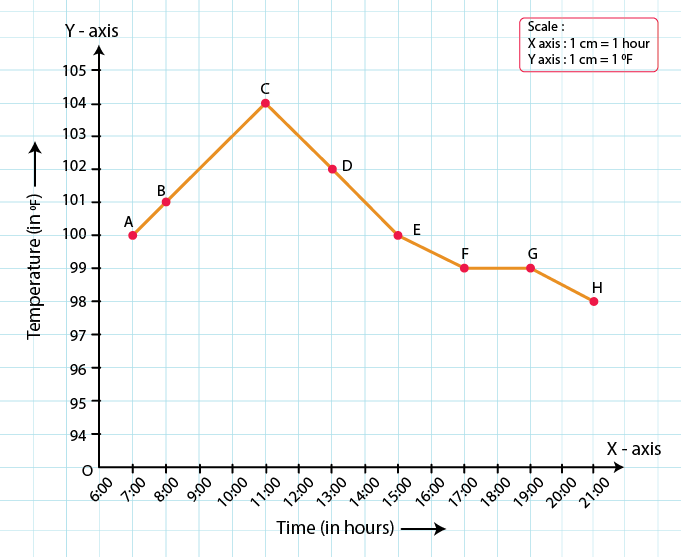(ii) We know that, time is an independent variable and temperature is a dependent variable. So, we take time on the x-axis and temperature on the y-axis.

Let us choose the following scale:

On x-axis: 2 cm = 2 hours

On y-axis: 1 cm = 1°F

Let us assume that on the x-axis, the coordinate of origin is 6:00.

On y-axis, the coordinate of origin is 94°F.

So, the coordinates of 0 are (6:00, 94).

Now, let us plot (8:00, 100), (10:00, 101), (12:00, 104) ….. (20:00, 100).

These points are joined to get the graphs representing the given information as shown in the figures below.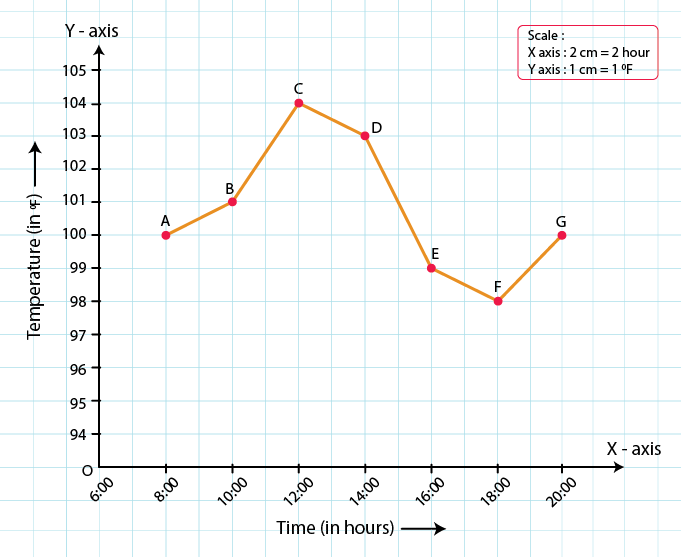7. Draw the velocity-time graph from the following data:

 Time (in hours) 7:00 8:00 9:00 10:00 11:00 12:00 13:00 14:00 Speed (in km/hr) 30 45 60 50 70 50 40 45

Solution:

We know that, time is an independent variable and speed is a dependent variable. So, we take time on the x-axis and speed on the y-axis.

By choosing the following scale:

On x-axis: 2 big division = 1 hour

On y-axis: 1 big division = 10 km/hr

Let us assume that on the x-axis, the coordinate of origin (O) is 7:00.

So, the coordinates of O are (7:00, 0).

Now, let us plot (7:00, 30), (8:00, 45), (9:00, 60), (10:00, 50), (11:00, 70), (12:00, 50), (13:00, 40), (14:00, 45).

These points are joined to get the graph representing the given information as shown in the figure below.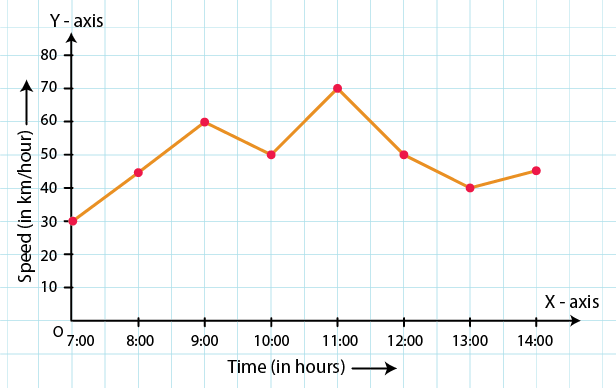8. The runs scored by a cricket team in first 15 overs are given below:

 Overs: I II III IV V VI VII VIII IX X XI XII XIII XIV XV Runs: 2 1 4 2 6 8 10 21 5 8 3 2 6 8 12

Draw the graph representing the above data in two different ways as a graph and as a bar chart.

Solution:

We know that, over is an independent variable and run is a dependent variable. So, we take overs on the x-axis and runs on the y-axis.

By choosing the following scale:

On x-axis: 1 cm = 1 over

On y-axis: 1 cm = 2 runs

Now, let us plot (I, 2), (II, 1), (III, 4)… (XV, 12).

These points are joined to get the graph representing the given information as shown in the figure below.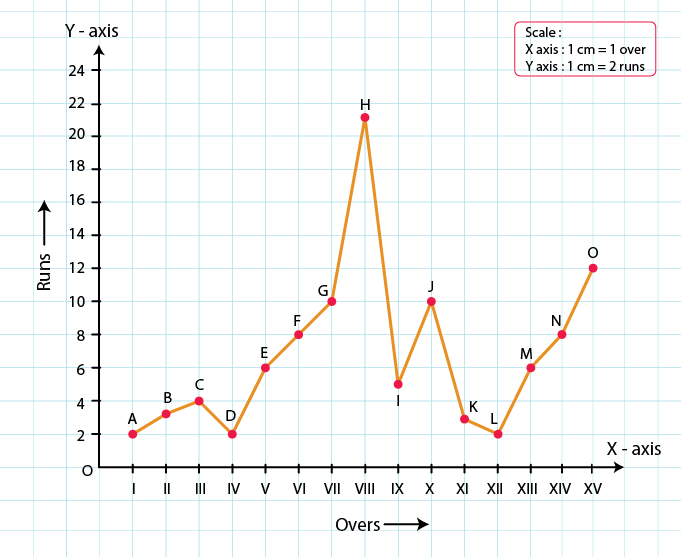The bar graph is as follows: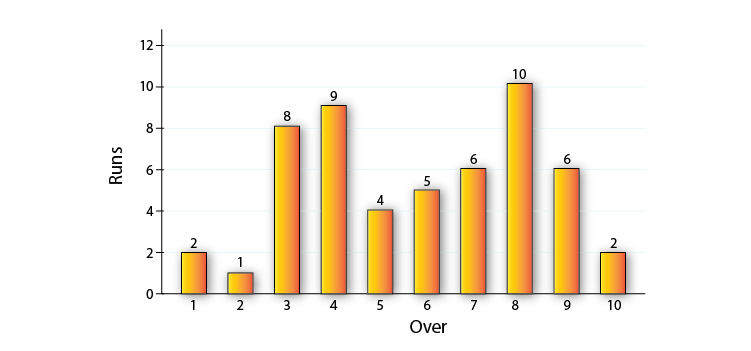9. The runs scored by two teams A and B in first 10 overs are given below:

 Overs I II III IV V VI VII VIII IX X Team A 2 1 8 9 4 5 6 10 6 2 Team B 5 6 2 10 5 6 3 4 8 10

Draw a graph depicting the data, making the graphs on the same axes in each case in two different ways as a graph and as a bar chart.

Solution:

We know that, over is an independent variable and run is a dependent variable. So, we take overs on x-axis and runs on the y-axis.

By choosing the following scale:

On x-axis: 1 cm = 1 over

On y-axis: 1 cm = 1 run

Now, let us plot (I, 2), (II, 1), (III, 8)… (X, 2) for team A and (I, 5), (II, 6), (III, 8) ….. (X, 10) for team B.

These points are joined to get the graph representing the given information as shown in the figure below.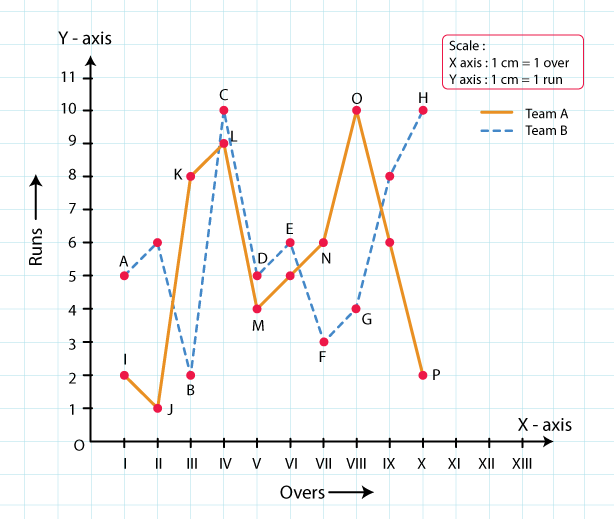The bar graph is as follows: